HTML-CSS-JS参考手册

# HTML

## HTML结构

``````<!DOCTYPE html>
<html>
<meta charset="UTF-8">
<!--移动端适配代码-->
<meta name="viewport" content="width=device-width, initial-scale=1, maximum-scale=1, minimum-scale=1, user-scalable=no">
<title>首页</title>
<body>
</body>
</html>``````

## 基本标签

div 布局容器
h1-h6 标题标签（h1权重最高 (页面中 最重要的位置logo 新闻标题)页面中只能出现一次），h3常用
p 段落标签
br 换行标签
hr 水平分割线标签
b 文本加粗
strong 文本加粗[强调]
i 文本斜体
em 文本斜体
s 文本删除线
del 文本删除线[强调]
u 文本下划线
ins 文本下划线[强调]
big 文本放大
small 文本缩小
sub 下标
sup 上标
pre 预格式文本[原样输出]
textarea 文本域
meta 单标签

&nbsp ； 空格
&lt ; <
&gt ; >

## 块级元素和行内元素

``````div
p
h1-h6
ul+li  ol+li  dl+dt+dd
hr``````

• 独占一行
• 可设置宽高，宽度默认100%，高度由内容决定（可参考父级高度设置百分比，如 height：100%）
• 可设置内外边距
• 可容纳行内元素和块级元素

``a   span   strong   b   em   i   sub   sup   u ``

• 和相邻行内元素在一行上

• 不能设置宽高，高度由内容决定（可通过line-height来设置高度）

• 只能容纳文本或行内元素

• 不单独占满一行，可以看成是能够在一行里进行左右排列的块元素
• 可设置宽高（如果元素没有设置宽高，则宽高由内容决定）
• 可设置内外边距

## 行内和块级元素转换

1. display

``````display: block; /* 行内元素转块级元素 */

display: inline; /* 块级元素转行内元素 */

display: inline-block; /* 行内块元素 */``````

2. float

``````float: left;
/* 或者 */
float: right;``````

3. position

``````position: absolute;
/* 或者 */
position: fixed;``````

# CSS

## 盒子模型

CSS的盒子模型有两种：

• 标准W3C盒子模型，如下图：``内容(content)    内边距(padding)    边框(border)    外边距(margin)``

``````* {
margin: 0;
}``````

``````#box {
width: 70px;
margin: 10px;
}``````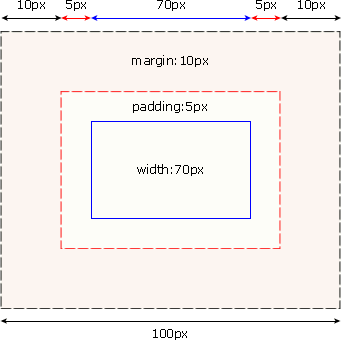1.元素空间大小

``````元素空间宽度 = width+ padding + margin + border

2.元素实际大小

``````元素实际宽度 = width+ padding + margin

``````box-sizing: content-box;     盒子大小 = width + padding + border（默认值）
box-sizing: border-box;        盒子大小 =  width（就是说  padding 和 border 是包含到width里面的）``````

## 选择器

### CSS选择器

#### 基本选择器

1 div、p 元素选择器 p { color: red }
2 #id id选择器 #info { color: red }
3 .class 类名选择器 .name { color: red }
4 * 通配符选择器 * { margin: 0; padding: 0;}

#### 多元素的组合选择器

1 div,p .one, p 多元素选择器 div,p { color: red }
2 div p .one p 后代选择器 .one p { color: red }
3 div>p .one>p 子元素选择器 .one p { color: red }
4 div+p .one+p 相邻元素选择器 .one+p { color: red }
``````交集选择器 (div.one,  p.red)

#### 属性选择器

1 E[att] 匹配所有具有att属性的E元素（E在此处可以省略，以下同）
2 E[att=val] 匹配所有att属性等于”val”的E元素
3 E[att~=val] 匹配所有att属性具有多个空格分隔的值、其中一个值等于”val”的E元素
4 E[att|=val] 匹配所有att属性具有多个连字号分隔（hyphen-separated）的值、其中一个值以”val”开头的E元素，主要用于lang属性，比如”en”、”en-us”、”en-gb”等等

[attr]

``````[title] {
color:red;
}``````

``````<h3 title="Hello world">Hello world</h3>
<a title="W3School" href="http://w3school.com.cn">W3School</a>``````

[attr=val]

``````[title=W3School] {
color:red;
}``````

``````<h3 title="W3School">W3School</h3>
<a title="W3School" href="http://w3school.com.cn">W3School</a>``````

[attr~=val]

``````[title~=hello] {
color:red;
}``````

``````<h3 title="hello world">Hello world</h3>
<p title="student hello">Hello W3School students!</h1>``````

[attr|=val]

``````[lang|=en] {
color:red;
}``````

``````<p lang="en">Hello!</p>
<p lang="en-us">Hi!</p>``````

#### 伪类

1 E:first-child 匹配父元素的第一个子元素
3 E:visited 匹配所有已被点击的链接
4 E:active 匹配鼠标已经其上按下、还没有释放的E元素
5 E:hover 匹配鼠标悬停其上的E元素
6 E:focus 匹配获得当前焦点的E元素
7 E:lang(c) 匹配lang属性等于c的E元素

#### 伪元素

1 E:first-line 匹配E元素的第一行
2 E:first-letter 匹配E元素的第一个字母
3 E:before 在E元素之前插入生成的内容
4 E:after 在E元素之后插入生成的内容

### CSS3选择器

#### CSS3同级元素通用选择器

1 E ~ F 匹配任何在E元素之后的同级F元素 p ~ .one { color: red }

#### CSS3属性选择器

1 E[att^=”val”] 属性att的值以”val”开头的元素
2 E[att\$=”val”] 属性att的值以”val”结尾的元素
3 E[att*=”val”] 属性att的值包含”val”字符串的元素

#### CSS3中与用户界面有关的伪类

1 E:enabled 匹配表单中激活的元素
2 E:disabled 匹配表单中禁用的元素
4 E::selection 匹配用户当前选中的元素

#### CSS 3中的结构性伪类

1 E:root 匹配文档的根元素，对于HTML文档，就是HTML元素
2 E:nth-child(n) 匹配其父元素的第n个子元素，第一个编号为1
3 E:nth-last-child(n) 匹配其父元素的倒数第n个子元素，第一个编号为1
4 E:nth-of-type(n) 与:nth-child()作用类似，但是仅匹配使用同种标签的元素
5 E:nth-last-of-type(n) 与:nth-last-child() 作用类似，但是仅匹配使用同种标签的元素
6 E:last-child 匹配父元素的最后一个子元素，等同于:nth-last-child(1)
7 E:first-of-type 匹配父元素下使用同种标签的第一个子元素，等同于:nth-of-type(1)
8 E:last-of-type 匹配父元素下使用同种标签的最后一个子元素，等同于:nth-last-of-type(1)
9 E:only-child 匹配父元素下仅有的一个子元素，等同于:first-child:last-child或 :nth-child(1):nth-last-child(1)
10 E:only-of-type 匹配父元素下使用同种标签的唯一一个子元素，等同于:first-of-type:last-of-type或 :nth-of-type(1):nth-last-of-type(1)
11 E:empty 匹配一个不包含任何子元素的元素，注意，文本节点也被看作子元素

p:nth-child(n)

``````<style>
p:nth-child(2) { background-color: red; }
</style>
<body>
<h1>这是标题</h1>
<p>第一个段落（背景红色***）</p>
<p>第二个段落</p>
<p>第三个段落</p>
<p>第四个段落</p>
</body>``````

p:first-child

``````<style>
p:first-child { background-color: red; }
</style>
<body>
<p>这个段落是其父元素（body）的首个子元素（背景红色***）</p>
<h1>欢迎访问我的主页</h1>
<p>这个段落不是其父元素的首个子元素</p>
<div>
<p>这个段落是其父元素（div）的首个子元素（背景红色***）</p>
<p>这个段落不是其父元素的首个子元素</p>
</div>
<p>你好</p>
</body>``````

p:nth-of-type(2)

``````<style>
p:nth-of-type(2) { background-color: red; }
</style>

<body>
<h1>这是标题</h1>
<h3>这是标题</h3>
<p>第一个段落</p>
<p>第二个段落（背景红色***）</p>
<p>第三个段落</p>
<p>第四个段落</p>
<p>第五个段落</p>
</body>``````

p:first-of-type

``````<style>
p:first-of-type { background-color: red; }
</style>

<body>
<h1>这是标题</h1>
<p>这是第一个段落（背景红色***）</p>
<p>这是第二个段落</p>
<p>这是第三个段落</p>
<p>这是第四个段落</p>
</body>``````

p:only-of-type

``````<style>
p:only-of-type { background-color: red; }
</style>

<body>
<div>
<p>这是一个段落（背景红色***）</p>
</div>
<div>
<p>这是一个段落</p>
<p>这是一个段落</p>
</div>
</body>``````

p:only-child

``````<style>
p:only-child { background-color: red; }
</style>

<body>
<div>
<p>这是一个段落（背景红色***）</p>
</div>
<div>
<p>这是一个段落</p>
<span>这是一个 span</span>
<p>这是一个段落</p>
</div>
</body>``````

#### CSS3的反选伪类

1 E:not(s) 匹配不符合当前选择器的任何元素 :not(p) { color: red }

#### CSS3中的 :target 伪类

1 E:target 匹配文档中特定”id”点击后的效果
``````:target {
color: red;
font-size: 30px;
}``````

E::before和E::after

``````div::before {
content:"开始";
}
div::after {
content:"结束";
}``````

E:after、E:before 在旧版本里是伪元素，CSS3的规范里“:”用来表示伪类，“::”用来表示伪元素，但是在高版本浏览器下E:after、E:before会被自动识别为E::after、E::before，这样做的目的是用来做兼容处理。

“:” 与 “::” 区别在于区分伪类和伪元素

## CSS优先级

``````优先级为:
!important  >  id  >  class  >  元素标签（element）
!important 比内联优先级高``````

## CSS继承性

``````字体相关：font-size、font-weight、font-style、font-family 等

``border、padding、margin、width、height``

## CSS单位

px 像素 (1px = 1/96th of 1in)

em 它是描述相对于应用在当前元素的字体尺寸，所以它也是相对长度单位。一般浏览器字体大小默认为16px，则2em == 32px；
ex 依赖于英文字母小 x 的高度
ch 数字 0 的宽度
rem 根元素（html）的 font-size
vw viewpoint width，视窗宽度，1vw=视窗宽度的1%
vh viewpoint height，视窗高度，1vh=视窗高度的1%
vmin vw和vh中较小的那个。
vmax vw和vh中较大的那个。
% 相对于父元素的长度、宽度百分比

## CSS定位

CSS的定位机制共3种： `文档流`(标准流)、`浮动``定位`

``````文档流：根据便签样式，自左往右，自上而下显示

static：主要用来清除定位
relative：相对定位，占位置，不脱标
abosolute：绝对定位，不占位置，脱标
fixed: 固定定位，相对于浏览器窗口进行定位

position 描述
static 自动定位（默认定位方式）
relative 相对定位，相对于其原文档流的位置进行定位
absolute 绝对定位，相对于其上一个已经定位的父元素进行定位
fixed 固定定位，相对于浏览器窗口进行定位

### 浮动

``````浮动脱离标准流，不占位置，脱标，浮动只有左右浮动

float: left; 浮动
none：默认值。元素不浮动，并会显示在其在文本中出现的位置
left：元素向左浮动
right：元素向右浮动
inherit：规定应该从父元素继承 float 属性的值``````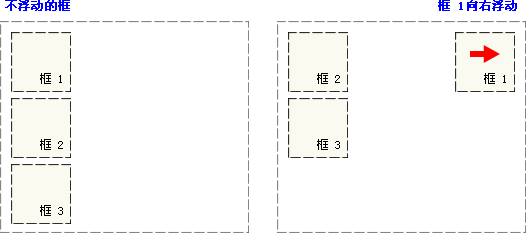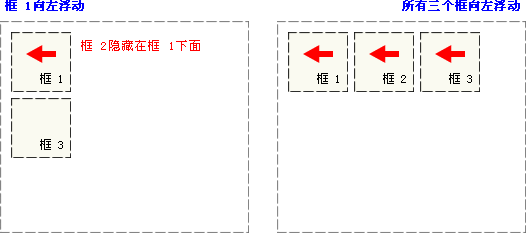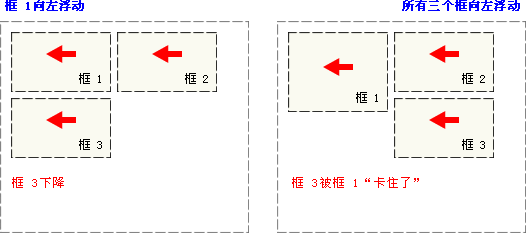### 清除浮动``````clear: both; 左侧和右侧均不允许出现浮动元素
none：默认值。允许浮动元素出现在两侧
left：在左侧不允许浮动元素
right：在右侧不允许浮动元素
both：在左右两侧均不允许浮动元素
inherit：规定应该从父元素继承 clear 属性的值``````

1、额外标签法

``在浮动元素末尾添加<div style=“clear:both”></div>``

2、父级元素添加overflow属性

``给父级添加 overflow:hidden / auto / scroll``

3、使用after伪元素

``````.clearfix:after{
content:"";
display:block;
height:0;
clear:both; /*额外标签法*/
visibility:hidden; /* 隐藏盒子 */
}
.clearfix{
*zoom:1; /*兼容IE6，IE7，IE6清楚浮动的方式 */
}``````

``使用：父元素添加类：class="clearfix"``

4、双伪元素法，推荐使用

``````.clearfix:before, .clearfix:after{
content:"";
display:table:
}
.clearfix:after{
clear:both;
}
.clearfix{
*zoom:1;
} ``````
``使用：父元素添加类：class="clearfix"``

### 相对定位

`设置为相对定位的元素框会偏移某个距离。元素仍然保持其未定位前的形状，它原本所占的空间仍保留`

``````.box2 {
position: relative;
left: 30px;
top: 20px;
}``````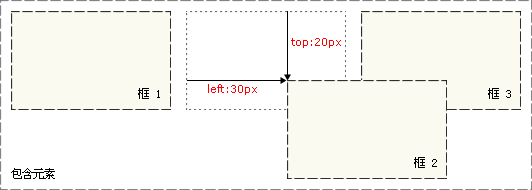### 绝对定位

`设置为绝对定位的元素框从文档流完全删除，并相对于其包含块定位，包含块可能是文档中的另一个元素或者是初始包含块。元素原先在正常文档流中所占的空间会关闭，就好像该元素原来不存在一样。元素定位后生成一个块级框，而不论原来它在正常流中生成何种类型的框`

``````.box2 {
position: absolute;
left: 30px;
top: 20px;
}``````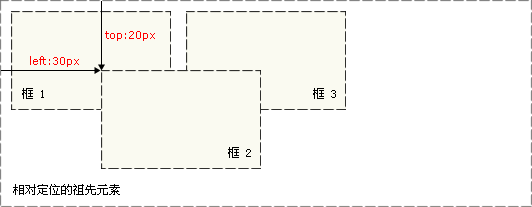## 外边距合并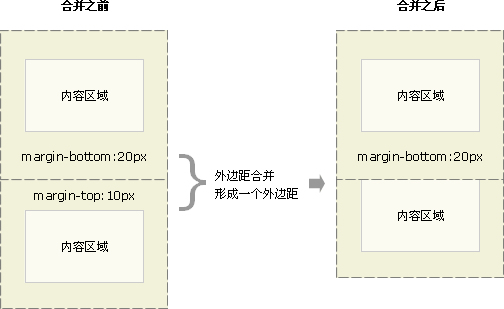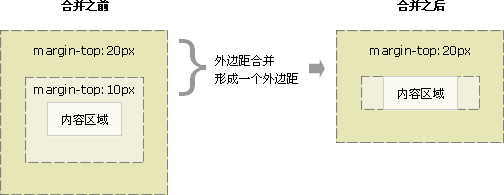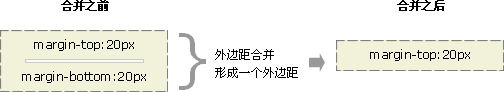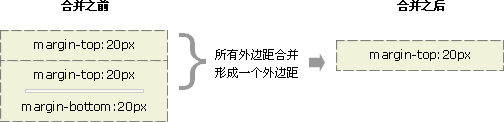• 可以为父元素定义1像素的上边框或上内边距
• 可以为父元素添加`overflow:hidden`
``````兄弟和兄弟之间    垂直方向相遇
1.浮动 定位特性
2.给父级 overflow:hidden; 溢出的处理  hidden隐藏  bfc
3.给父级 display:inline-block;``````

## BFC(块级格式化上下文)

BFC块级格式化上下文，是一个独立的布局环境，其中的元素布局是不受外界的影响

​ 块级元素：父级（块元素）

​ 内容：子元素（块元素）

​ 其他元素：与内容同级别的兄弟元素

``````1. float属性不为none
2. position为absolute或fixed
3. display为inline-block, table-cell, table-caption, flex, inline-flex
4. overflow不为visible( hidden、scroll、auto)``````

BFC布局规则特性

• 在BFC中，内部的盒子会在垂直方向，一个接一个地排列

• 在BFC中，盒子垂直方向的距离由margin决定，属于同一个BFC的两个相邻盒子的margin会发生重叠

• 在BFC中，每一个盒子（块盒与行盒）的左外边缘（margin-left）会触碰到容器的左边缘(border-left)，对于从左往右的格式化，否则相反，即使存在浮动也是如此

• BFC的区域不会与浮动盒子产生交集，而是紧贴浮动盒子边缘

• BFC就是页面上的一个隔离的独立容器，容器里面的子元素不会影响到外面的元素。反之也如此

• 计算BFC的高度时，自然也会检测浮动或者定位的盒子高度

BFC的主要作用

• 利用BFC避免垂直margin重叠
• 清除元素内部浮动
• 自适应右侧盒子布局

`利用BFC避免垂直margin重叠`

``````<style>
p {
color: #f55;
background: #fcc;
width: 200px;
line-height: 100px;
text-align: center;
margin: 100px;
}
</style>
<body>
<p>Haha</p>
<p>Hehe</p>
</body>``````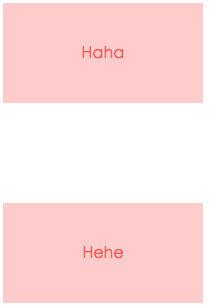``````<style>
p {
color: #f55;
background: #fcc;
width: 200px;
line-height: 100px;
text-align: center;
margin: 100px;
}
.wrap {
overflow: hidden;
}
</style>
<body>
<p>Haha</p>
<div class="wrap">
<p>Hehe</p>
</div>
</body>``````

`清除元素内部浮动`

``````<style>
.parent {
border: 5px solid #fcc;
width: 300px;
}

.child {
border: 5px solid #f66;
width: 100px;
height: 100px;
float: left;
}
</style>
<body>
<div class="parent">
<div class="child"></div>
<div class="child"></div>
</div>
</body>``````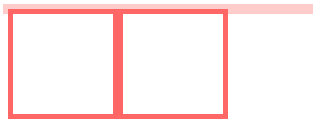``````.parent {
overflow: hidden;
}``````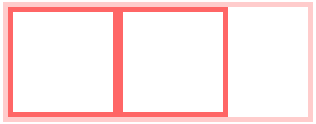`自适应右侧盒子布局`

``````<style>
.box {
width: 300px;
position: relative;
}

.left {
width: 100px;
height: 150px;
float: left;
background: #f66;
}

.right {
height: 200px;
background: #fcc;
}
</style>

<body>
<div class="box">
<div class="left"></div>
<div class="right"></div>
</div>
</body>``````BFC规则：

​ 在BFC中，每一个盒子（块盒与行盒）的左外边缘（margin-left）会触碰到容器的左边缘(border-left)，对于从左往右的格式化，否则相反，即使存在浮动也是如此

​ BFC的区域不会与浮动盒子产生交集，而是紧贴浮动盒子边缘

``````.right {
overflow: hidden;
}``````BFC布局与普通文档流布局区别

``````1. 浮动的元素是不会被父级计算高度
2. 非浮动元素会覆盖浮动元素的位置
3. margin会传递给父级
4. 两个相邻元素上下margin会重叠``````

BFC布局规则

``````1. 浮动的元素会被父级计算高度（父级触发了BFC）
2. 非浮动元素不会覆盖浮动元素位置（非浮动元素触发了BFC）
3. margin不会传递给父级（父级触发了BFC）
4. 两个相邻元素上下margin不会重叠（给其中一个元素增加一个父级，然后让他的父级触发BFC）           ``````

## IFC(行级格式化上下文)

IFC布局规则

``````1. 内联元素会在水平方向一个个地放置
2. IFC地高度是由最高盒子的高度决定地
3. 当一行不够放置的时候会自动切换到下一行

## CSS3-flex布局

### flex 简介

​ 布局的传统解决方案，基于盒状模型，依赖 display 属性 + position属性 + float属性。它对于那些特殊布局非常不方便，比如，垂直居中就不容易实现。

​ CSS3在布局方面做了非常大的改进，使得我们对块级元素的布局排列变得十分灵活，适应性非常强，其强大的伸缩性，在响应式开中可以发挥极大的作用。

​ 2009年，W3C 提出了一种新的方案—-Flex 布局，可以简便、完整、响应式地实现各种页面布局。目前，它已经得到了所有浏览器的支持，这意味着，现在就能很安全地使用这项功能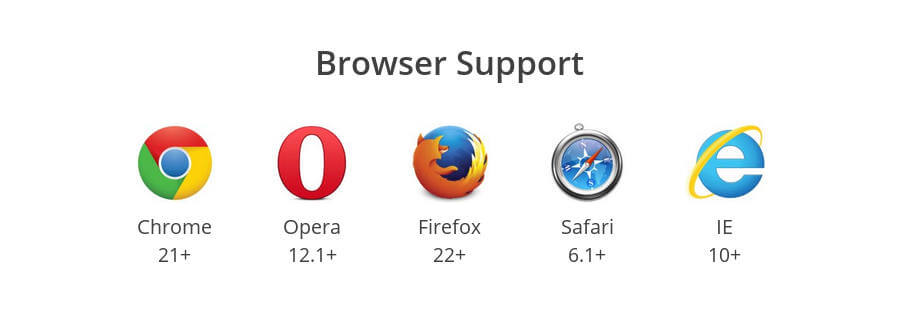Flex 是 Flexible Box 的缩写，意为`弹性布局`，用来为盒状模型提供最大的灵活性。

``````.box{
display: flex;
}``````

``````.box{
display: inline-flex;
}``````

Webkit 内核的浏览器，必须加上-webkit前缀

``````.box{
display: -webkit-flex; /* Safari */
display: flex;
}``````

### 基本概念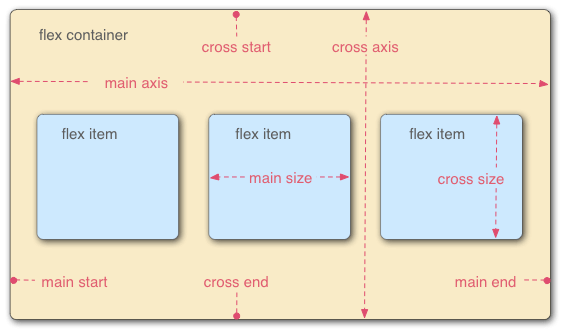### 容器属性

#### 一个简单的例子

``````<!DOCTYPE html>
<html lang="en">
<meta charset="UTF-8">
<title>flex布局</title>
<style>
* {
margin: 0;
}
.box {
width: 500px;
height: 200px;
background-color: #ccc;
color: #666;
margin: 0 auto;
/* 设置父容器的为伸缩盒子 */
display: flex;
/* 设置子元素在主轴方向上的排列方式 */
/* justify-content: flex-start; */
}
.left {
flex: 1; /* flex是用来设置当前伸缩子项占据剩余空间的比例值 */
height: 200px;
background-color: pink;
}
.right {
flex: 4;
height: 200px;
background-color: skyblue;
}
</style>
<body>
<div class="box">
<div class="left">flex: 1=100</div>
<div class="right">flex: 4=400</div>
</div>
</body>
</html>``````#### flex-direction

`flex-direction`属性决定主轴的方向

``````.box {
flex-direction: row | row-reverse | column | column-reverse;
}

row（默认值）：主轴为水平方向，起点在左端。
row-reverse：主轴为水平方向，起点在右端。
column：主轴为垂直方向，起点在上沿。
column-reverse：主轴为垂直方向，起点在下沿。``````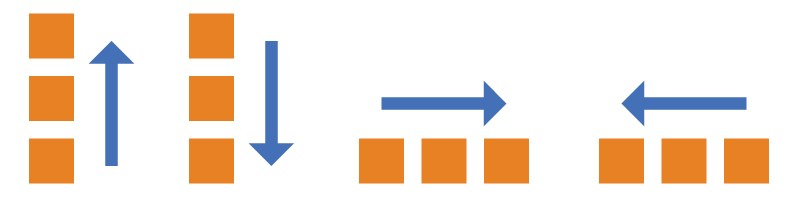#### flex-wrap

``````.box{
flex-wrap: nowrap | wrap | wrap-reverse;
}

nowrap（默认）：不换行，强制一行内显示
wrap：自动换行显示，第一行在上方
wrap-reverse:自动换行且反向``````

（1）nowrap（默认）：强制一行内显示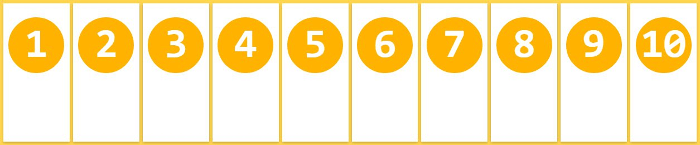（2）wrap：自动换行显示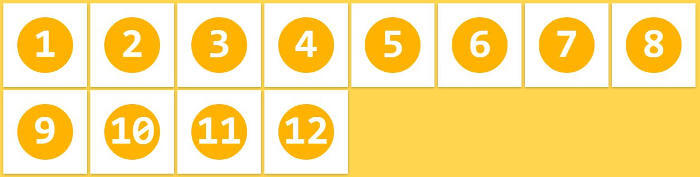（3）wrap-reverse:自动换行且反向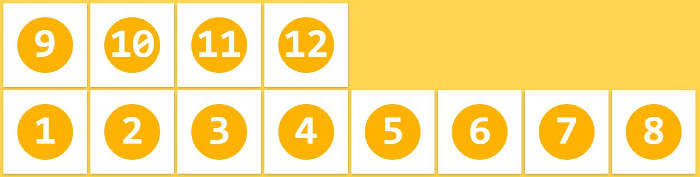#### flex-flow

`flex-flow`属性是flex-direction属性和flex-wrap属性的简写形式，默认值为row nowrap

``````.box {
flex-flow: <flex-direction> || <flex-wrap>;
}``````#### justify-content

`justify-content`属性定义了项目在主轴上的对齐方式

``````.box {
justify-content: flex-start | flex-end | center | space-between | space-around | space-evenly;
}

flex-start（默认值）：左对齐
flex-end：右对齐
center： 居中
space-between：两端对齐，项目之间的间隔都相等。
space-around：每个项目两侧的间隔相等。所以，项目之间的间隔比项目与边框的间隔大一倍。
space-evenly: 间隔相等``````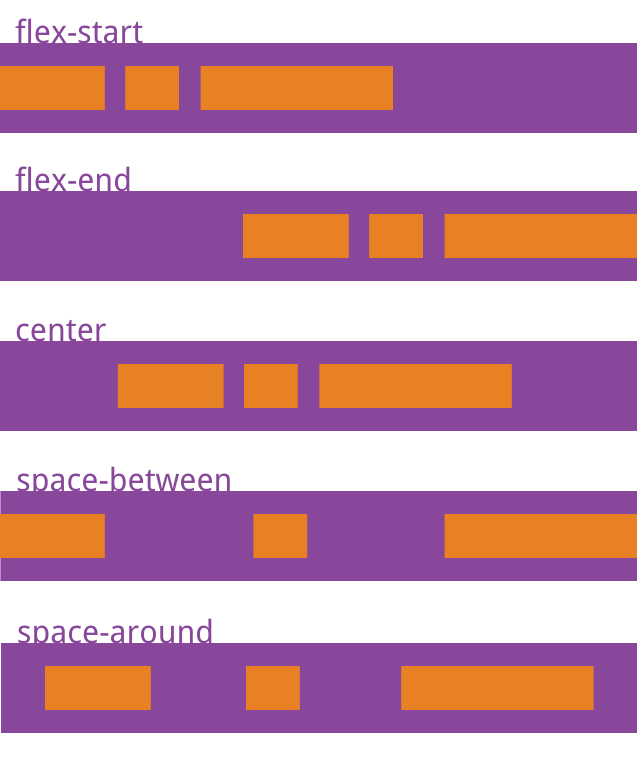#### align-items

`align-items`属性定义项目在交叉轴上如何对齐

``````.box {
align-items: flex-start | flex-end | center | baseline | stretch;
}

flex-start：交叉轴的起点对齐。
flex-end：交叉轴的终点对齐。
center：交叉轴的中点对齐。
baseline: 项目的第一行文字的基线对齐。
stretch（默认值）：如果项目未设置高度或设为auto，将占满整个容器的高度。``````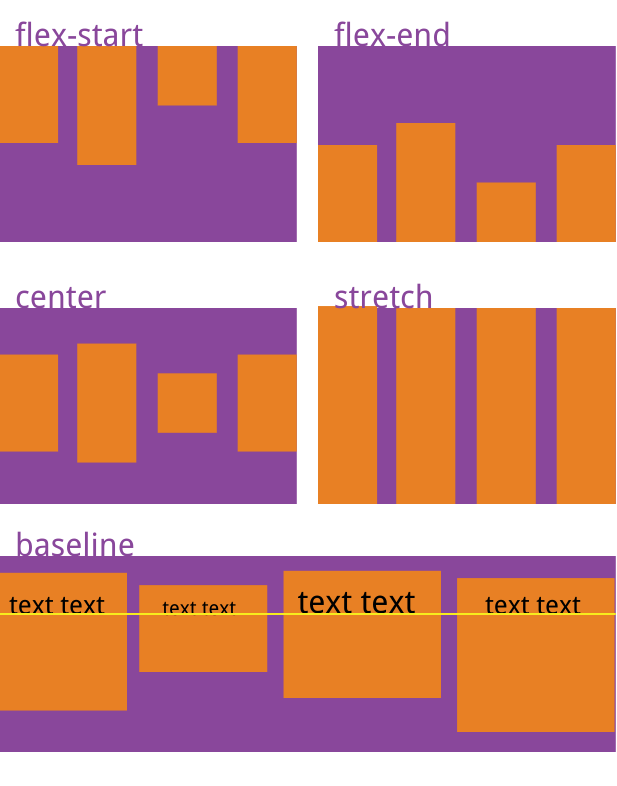#### align-content

`align-content`属性定义了多根轴线的对齐方式。如果项目只有一根轴线，该属性不起作用。

``````.box {
align-content: flex-start | flex-end | center | space-between | space-around | stretch;
}

flex-start：与交叉轴的起点对齐。
flex-end：与交叉轴的终点对齐。
center：与交叉轴的中点对齐。
space-between：与交叉轴两端对齐，轴线之间的间隔平均分布。
space-around：每根轴线两侧的间隔都相等。所以，轴线之间的间隔比轴线与边框的间隔大一倍。
stretch（默认值）：轴线占满整个交叉轴。``````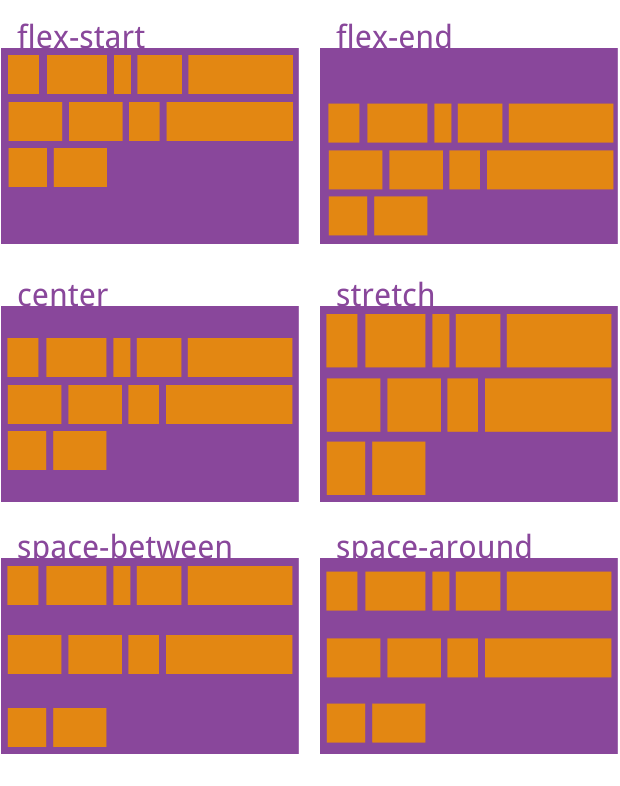### 项目属性

#### flex-grow

`flex-grow`属性定义项目的放大比例，默认为 0，即如果存在剩余空间，也不放大

``````.item {
flex-grow: <number>; /* default 0 */
}``````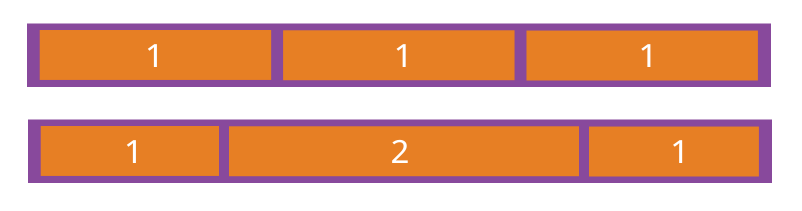#### flex-shrink

`flex-shrink`属性定义了项目的缩小比例，默认为1，即如果空间不足，该项目将缩小

``````.item {
flex-shrink: <number>; /* default 1 */
}``````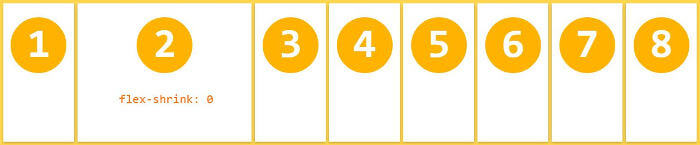#### flex basis

`flex-basis`属性定义了在分配多余空间之前，项目占据的主轴空间（main size）。浏览器根据这个属性，计算主轴是否有多余空间。它的默认值为`auto`，即项目的本来大小

``````.item {
flex-basis: <length> | auto; /* default auto */
}``````

#### flex属性

`flex`属性是`flex-grow`, `flex-shrink``flex-basis`的简写，默认值为`0 1 auto`。后两个属性可选。

``````.item {
flex: none | [ <'flex-grow'> <'flex-shrink'>? || <'flex-basis'> ]
}``````

#### align-self

`align-self`属性允许单个项目有与其他项目不一样的对齐方式，可覆盖`align-items`属性。默认值为`auto`，表示继承父元素的`align-items`属性，如果没有父元素，则等同于`stretch`

``````.item {
align-self: auto | flex-start | flex-end | center | baseline | stretch;
}``````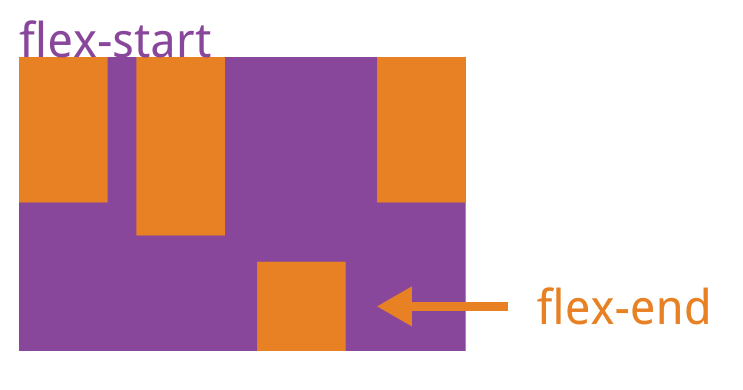## CSS 注意点

### line-height: 0 的情况

，中间三个搞定

``````

.father {
width: 100px;
margin: 50px auto;
line-height: 30px;
background-color: pink;
span {
display: inline-block;
width: 30px;
height: 30px;
background-color: blue;
}
}
````````````

1
1
1

````````````

.father {
width: 100px;
margin: 50px auto;
line-height: 30px;
background-color: pink;
line-height: 0;
span {
display: inline-block;
width: 30px;
height: 30px;
background-color: blue;
}
}
``````### font-size: 0 的情况

``````

1
2
3

.box {
width: 90px;
height: 60px;
margin: 50px auto;
border: 1px solid #ccc;
div {
display: inline-block;
width: 30px;
font-size: 14px;
box-sizing: border-box;
border: 1px solid green;
}
}
````````````
.box {
width: 90px;
height: 60px;
margin: 50px auto;
border: 1px solid #ccc;
font-size: 0;
div {
display: inline-block;
width: 30px;
font-size: 14px;
box-sizing: border-box;
border: 1px solid green;
}
}
``````## CSS技巧

### 长文本处理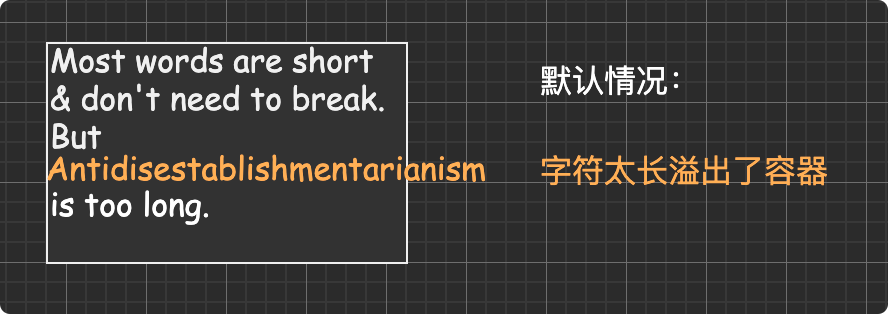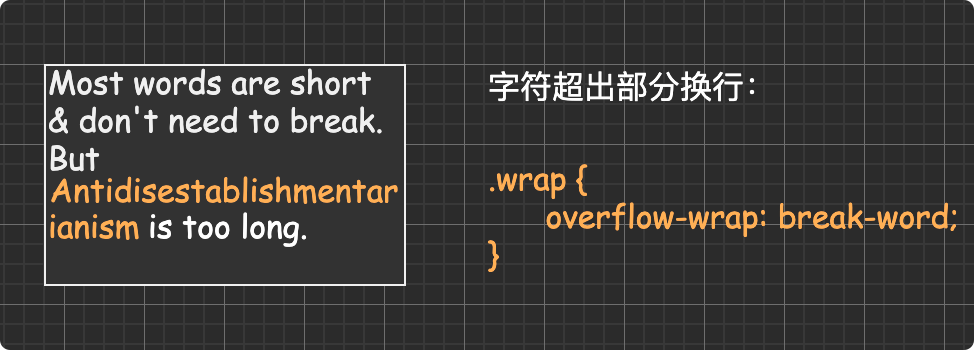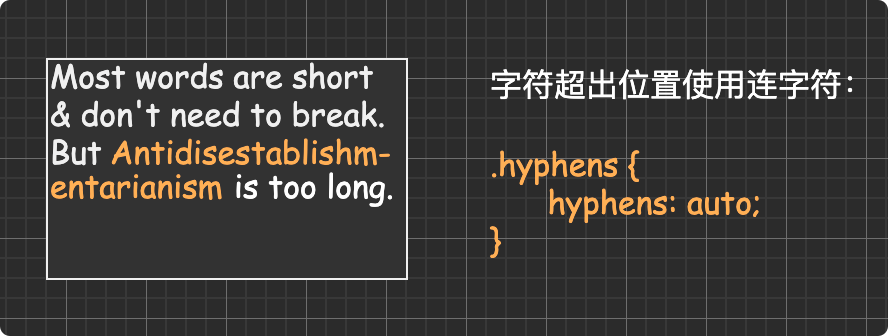### 文本超出省略

#### 单行文本超出省略

``````.line-1 {
overflow: hidden; /* 溢出隐藏 */
text-overflow: ellipsis; /* 超出部分显示 省略号 */
white-space: nowrap; /* 强制一行内显示 */
}``````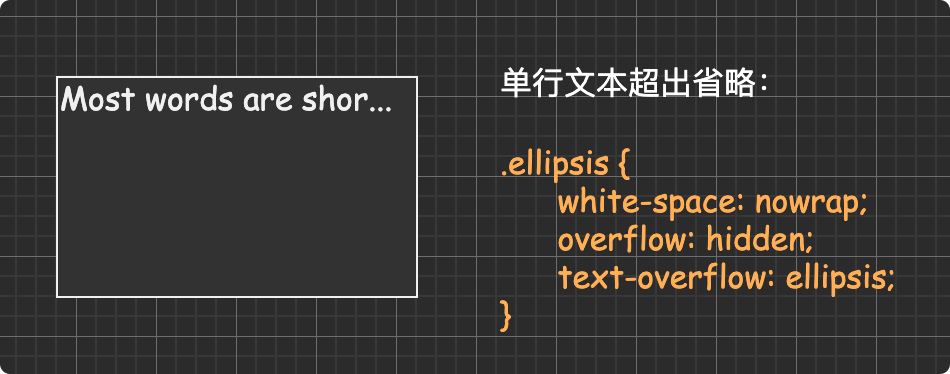#### 多行文本超出省略

``````.line-2 {
overflow: hidden;
text-overflow: ellipsis;
display: -webkit-box;
-webkit-line-clamp: 2;
-webkit-box-orient: vertical;
}``````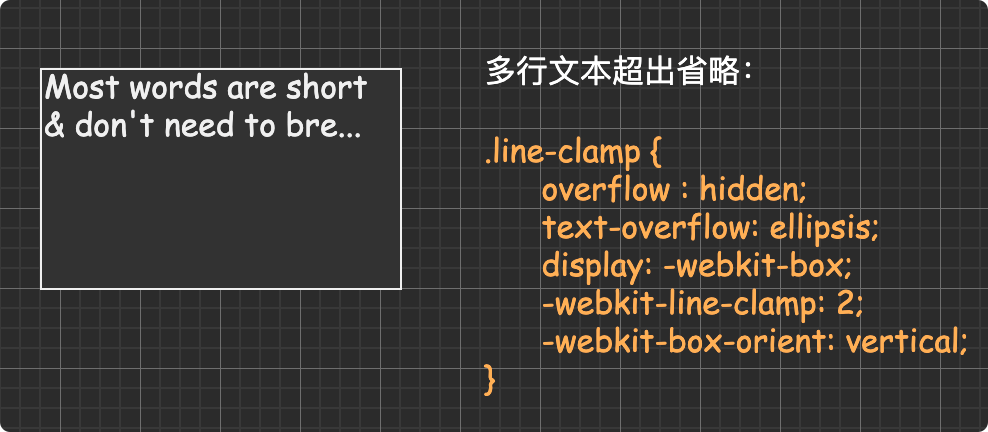### 水平垂直居中

#### 单行的文本、行内元素、行内块元素

``````.parent {
text-align: center;
}``````

``````.single-line {
}``````

``````.single-line {
height: 100px;
line-height: 100px;
}``````

5.CSS实现垂直水平居中（块级元素）———————————————————————————————

2.父元素相对定位，子元素绝对对位+`margin`负值

1. 定位+

``transform``

5.`flex`布局+`margin`

``````<style>
.father {
width: 500px;
height: 300px;
background: lightcoral;
display: flex;
}
.son {
background: lightblue;
margin: auto;
}
</style>
<div class="father">
<div class="son">我要垂直水平居中</div>
</div>``````

1

#### 固定宽高的块级盒子

``````.box {
width: 100px;
height: 100px;
background: red;
margin: 0 auto;
}``````

``````<style>
.box {
width: 500px;
height: 400px;
background-color: pink;
position: relative;
}

.son {
width: 200px;
height: 100px;
background-color: blue;
position: absolute;
top: 50%;
left: 50%;
margin-top: -50px;
margin-left: -100px;
}
</style>

<body>
<div class="box">
<div class="son"></div>
</div>
</body>``````
``````<style>
.box {
width: 500px;
height: 400px;
background-color: pink;
position: relative;
}

.son {
width: 200px;
height: 100px;
background-color: blue;
position: absolute;
left: 0;
right: 0;
top: 0;
bottom: 0;
margin: auto;
}
</style>

<body>
<div class="box">
<div class="son"></div>
</div>
</body>``````

#### 不固定宽高的块级盒子

``````<style>
.box {
width: 500px;
height: 400px;
background-color: pink;
position: relative;
}

.son {
width: 200px;
height: 100px;
background-color: blue;
position: absolute;
top: 50%;
left: 50%;
transform: translate(-50%, -50%);
}
</style>

<body>
<div class="box">
<div class="son"></div>
</div>
</body>``````

``````<style>
.box {
width: 500px;
height: 400px;
background-color: pink;
display: flex;
justify-content: center;
align-items: center;
}

.son {
width: 200px;
height: 100px;
background-color: blue;
}
</style>

<body>
<div class="box">
<div class="son"></div>
</div>
</body>``````

``````<style>
.box {
width: 500px;
height: 400px;
background-color: pink;
display: flex;
}

.son {
width: 200px;
height: 100px;
background-color: blue;
margin: auto;
}
</style>

<body>
<div class="box">
<div class="son"></div>
</div>
</body>``````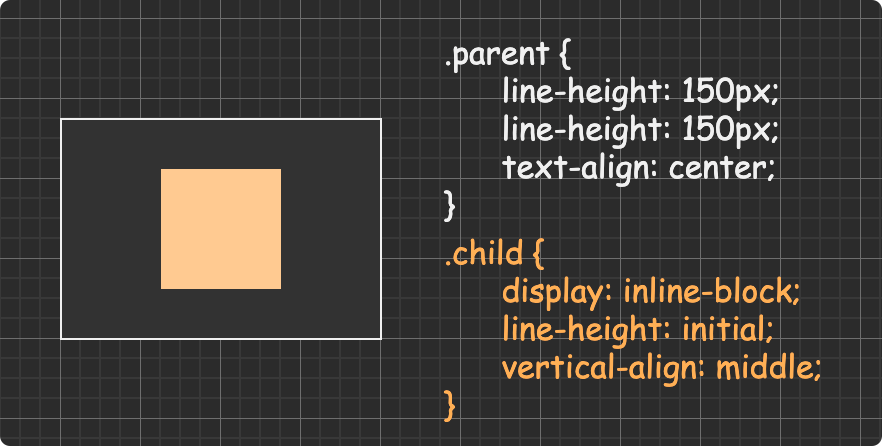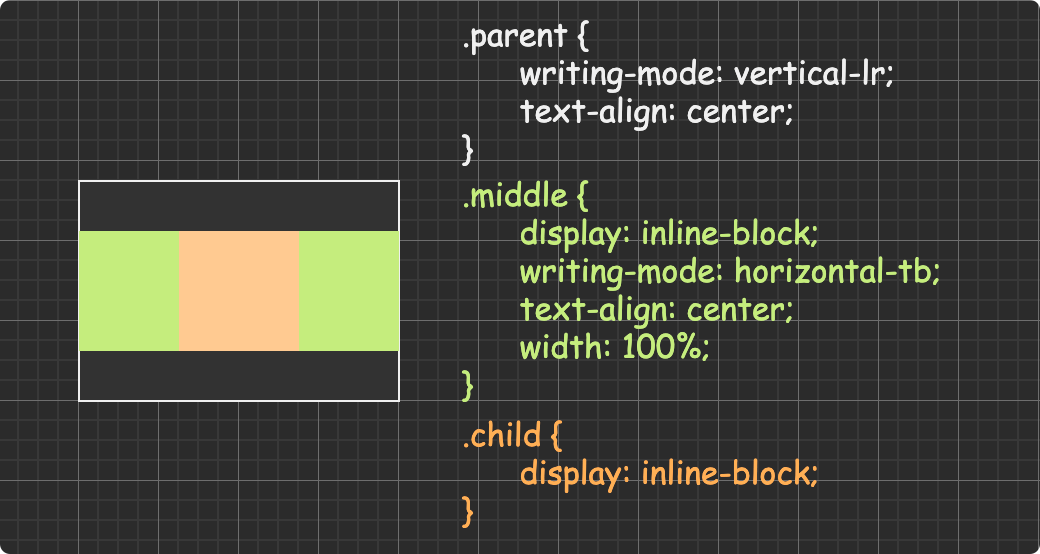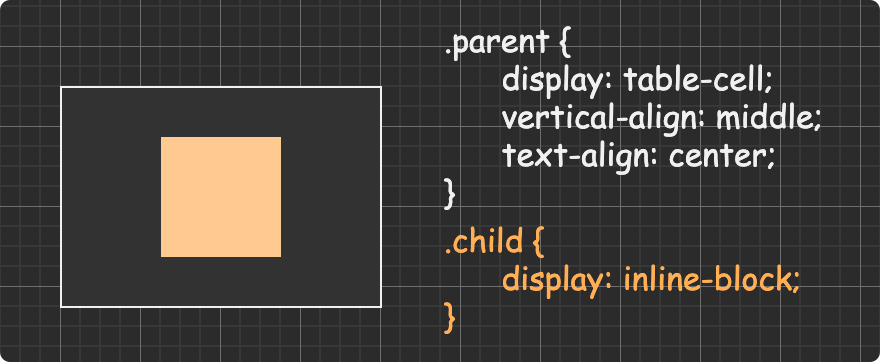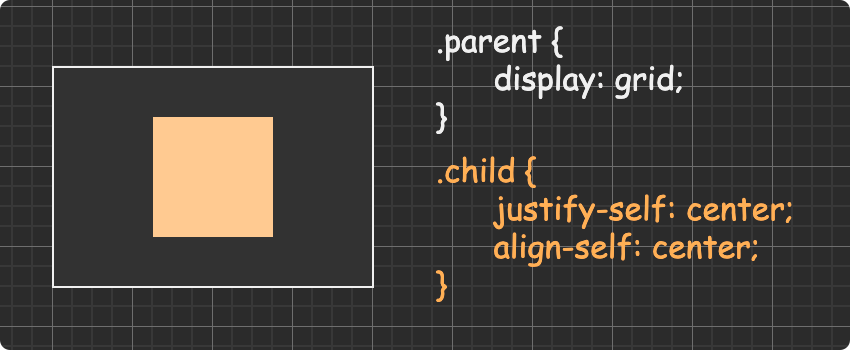display：table-cell 加上 vertical-align：middle 使高度不同的元素都垂直居中，其中div的display：inline-block使几个div在同一行显示。

``````<div class="parent">
<p class="son">
会议认为，党的十八大以来，我国经济发展取得历史性成就、
发生历史性变革，为其他领域改革发展提供了重要物质条件。经济实力
再上新台阶，经济年均增长7.1%，成为世界经济增长的主要动力源和稳定器。
</p>
</``````
``````.parent {
display: table;
width: 300px;
height: 300px;
text-align: center;
}
.son {
display: table-cell;
vertical-align: middle;
height: 200px;
background-color: yellow;
}``````

### 移动端1像素解决方案

``````/*手机端实现真正的一像素边框*/
.border-1px, .border-bottom-1px, .border-top-1px, .border-left-1px, .border-right-1px {
position: relative;
}

/*线条颜色 黑色*/
.border-1px::after, .border-bottom-1px::after, .border-top-1px::after, .border-left-1px::after, .border-right-1px::after {
background-color: #000;
}

/*底边边框一像素*/
.border-bottom-1px::after {
content: "";
position: absolute;
left: 0;
bottom: 0;
width: 100%;
height: 1px;
transform-origin: 0 0;
}

/*上边边框一像素*/
.border-top-1px::after {
content: "";
position: absolute;
left: 0;
top: 0;
width: 100%;
height: 1px;
transform-origin: 0 0;
}

/*左边边框一像素*/
.border-left-1px::after {
content: "";
position: absolute;
left: 0;
top: 0;
width: 1px;
height: 100%;
transform-origin: 0 0;
}

/*右边边框1像素*/
.border-right-1px::after {
content: "";
box-sizing: border-box;
position: absolute;
right: 0;
top: 0;
width: 1px;
height: 100%;
transform-origin: 0 0;
}

/*边框一像素*/
.border-1px::after {
content: "";
box-sizing: border-box;
position: absolute;
left: 0;
top: 0;
width: 100%;
height: 100%;
border: 1px solid gray;
}

/*设备像素比*/
/*显示屏最小dpr为2*/
@media (-webkit-min-device-pixel-ratio: 2) {
.border-bottom-1px::after, .border-top-1px::after {
transform: scaleY(0.5);
}

.border-left-1px::after, .border-right-1px::after {
transform: scaleX(0.5);
}

.border-1px::after {
width: 200%;
height: 200%;
transform: scale(0.5);
transform-origin: 0 0;
}
}

/*设备像素比*/
@media (-webkit-min-device-pixel-ratio: 3)  {
.border-bottom-1px::after, .border-top-1px::after {
transform: scaleY(0.333);
}

.border-left-1px::after, .border-right-1px::after {
transform: scaleX(0.333);
}

.border-1px::after {
width: 300%;
height: 300%;
transform: scale(0.333);
transform-origin: 0 0;
}
}
/*需要注意<input type="button">是没有:before, :after伪元素的*/``````

1. 给img vertical-align:middle | top等等。 让图片不要和基线对齐。

​ 2. 给img 添加 display：block; 转换为块级元素就不会存在问题了。

``````vertical-align:baseline;
1.图片下方间隙问题
解决方法 => 给img任意值覆盖baseline
2.图文的对齐方式  行内元素或者行内块元素之间的对齐方式
解决方法  顶部对齐  给两个元素同时加上vertical-align:top;
top 对齐元素(你设置了vertical的元素)的顶端 与line-box 的顶端对齐
bottom  对齐元素(你设置了vertical的元素)的底端 与line-box 的底端对齐
middle (常用)对齐元素 的中线 父元素的基线加上小写x``````

``````.wrap {
display: inline-block;
overflow: hidden
vertical-align: bottom
}``````

``````// 单行文本
.wrap {
overflow:hidden;/*超出部分隐藏*/
text-overflow:ellipsis;/*超出部分显示省略号*/
white-space:nowrap;/*规定段落中的文本不进行换行 */
}
// 多行文本
.wrap {
width: 100%;
overflow: hidden;
display: -webkit-box;   //将对象作为弹性伸缩盒子模型显示  *必须结合的属性*
-webkit-box-orient: vertical;   //设置伸缩盒对象的子元素的排列方式  *必须结合的属性*
-webkit-line-clamp: 3;   //用来限制在一个块元素中显示的文本的行数
word-break: break-all;   //让浏览器实现在任意位置的换行 *break-all为允许在单词内换行*
}``````

css实现不换行、自动换行、强制换行

``````//不换行
.wrap {
white-space:nowrap;
}
//自动换行
.wrap {
word-wrap: break-word;
word-break: normal;
}
//强制换行
.wrap {
word-break:break-all;
}``````

CSS实现文本两端对齐

``````.wrap {
text-align: justify;
text-justify: distribute-all-lines;  //ie6-8
text-align-last: justify;  //一个块或行的最后一行对齐方式
-moz-text-align-last: justify;
-webkit-text-align-last: justify;
}``````

``````// 单列展示时
.wrap {
width: 25px;
line-height: 18px;
height: auto;
font-size: 12px;
word-wrap: break-word;/*英文的时候需要加上这句，自动换行*/
}
// 多列展示时
.wrap {
height: 210px;
line-height: 30px;
text-align: justify;
writing-mode: vertical-lr;  //从左向右
writing-mode: tb-lr;        //IE从左向右
//writing-mode: vertical-rl;  -- 从右向左
//writing-mode: tb-rl;        -- 从右向左
}``````

``````.wrap {
// 如果按tab能选中该元素，如button，然后按回车还是能执行对应的事件，如click。
pointer-events: none;
cursor: default;
}``````

``````.wrap {
-webkit-touch-callout: none;
-webkit-user-select: none;
-khtml-user-select: none;
-moz-user-select: none;
-ms-user-select: none;
user-select: none;
}``````

cursor属性

``````.wrap {
cursor：pointer; // 小手指；
cursor：help; // 箭头加问号；
cursor：wait; // 转圈圈；
cursor：move; // 移动光标；
cursor：crosshair; // 十字光标
}``````

``````.wrap {
transform: translateZ(0);
}``````

``img {max-width: 100%}``

Text-transform和Font Variant

``````p {text-transform: uppercase}  // 将所有字母变成大写字母
p {text-transform: lowercase}  // 将所有字母变成小写字母
p {text-transform: capitalize} // 首字母大写
p {font-variant: small-caps}   // 将字体变成小型的大写字母``````

``````.wrap {
filter:alpha(opacity=50);
-moz-opacity:0.5;
-khtml-opacity: 0.5;
opacity: 0.5;
}``````

``````.wrap {
-webkit-transform-style: preserve-3d;
-webkit-backface-visibility: hidden;
-webkit-perspective: 1000;
}``````

``````overflow-y: scroll;

::-webkit-scrollbar {
width: 5px;
}

::-webkit-scrollbar-track {
background-color: #ffa336;
}

::-webkit-scorllbar-thumb {
background-color: #ffc076;
}``````

``````body {
white-space: pre-line;
}``````

``````.wrap {
border-color: transparent transparent green transparent;
border-style: solid;
border-width: 0px 300px 300px 300px;
height: 0px;
width: 0px;
}``````

``````a {outline: none}
a {outline: 0}``````

``a:after{content:" (" attr(href) ") ";}``

select内容居中显示、下拉内容右对齐

``````select{
text-align: center;
text-align-last: center;
}
select option {
direction: rtl;
}``````

``````input{
color:  #fff;
caret-color: red;
}``````

``````//webkit内核的浏览器
input::-webkit-input-placeholder {
color: #c2c6ce;
}
//Firefox版本4-18
input:-moz-placeholder {
color: #c2c6ce;
}
//Firefox版本19+
input::-moz-placeholder {
color: #c2c6ce;
}
//IE浏览器
input:-ms-input-placeholder {
color: #c2c6ce;
}``````

``````// 父元素下的子元素是行内元素
.wrap {
white-space: nowrap;
}
// 若父元素下的子元素是块级元素
.wrap {
white-space: nowrap;  // 子元素不被换行
display: inline-block;
}``````

``````.wrap {
height: 100,
line-height: 100
}
img {
vertival-align：middle
}
// vertical-align css的属性vertical-align用来指定行内元素（inline）或表格单元格（table-cell）元素的垂直对齐方式。只对行内元素、表格单元格元素生效，不能用它垂直对齐块级元素
// vertical-align：baseline/top/middle/bottom/sub/text-top;``````

``````        .scale {
width: 100%;
height: 0;
position: relative;
}

.item {
position: absolute;
width: 100%;
height: 100%;
background-color: 499e56;
}
<div class="scale">
<div class="item">
这里是所有子元素的容器
</div>
</div>``````

transfrom的rotate属性在span标签下失效

``````span {
display: inline-block
}``````

``````    .wrap {
width: 200px;
height: 200px;
color: #000;
font-size: 30px;
border: 50px solid currentColor;
// border: 50px solid; // 实现二
}``````

``````// 复选框
input {
&.checkbox {
-webkit-appearance: none;
width: 14px !important;
height: 14px !important;
background: url(../img/checkbox14.png) no-repeat;
cursor: pointer;
&:checked {
background: url(../img/checkboxed14.png) no-repeat;
}
}
}``````

``````.box{
position: absolute;
top: 50%;
-webkit-transform: translateY(-50%);
-o-transform: translateY(-50%);
transform: translateY(-50%);
}``````

``````button {
background-size: auto 200%;
background-position: 0 100%;
transition: background-position 0.5s;
}
button:hover {
background-position: 0 0;
}``````

``````td {
white-space: nowrap;
}``````

``````h1 {
text-indent: -9999px;
margin: 0 auto;
width: 320px;
height: 85px;
background: transparent url("images/logo.png") no-repeat scroll;
}``````

``````.container {
min-height: 6.5em;
display: table-cell;
vertical-align: middle;
} ``````

CSS固定页脚

``````#footer {
position: fixed;
left: 0px;
bottom: 0px;
height: 30px;
width: 100%;
background: #444;
}
/* IE 6 */
* html #footer {
position: absolute;
top: expression((0-(footer.offsetHeight)+(document.documentElement.clientHeight ? document.documentElement.clientHeight : document.body.clientHeight)+(ignoreMe = document.documentElement.scrollTop ? document.documentElement.scrollTop : document.body.scrollTop))+'px');
}``````

# JavaScript## 简介

JavaScript是一门脚本语言、解释性语言、动态类型的语言、基于对象的语言

JavaScript分为三个部分

`````` 1. ECMAScript 标准                 js的基本的语法
2. DOM (Document Object Model)       文档对象模型
3. BOM (Browser Object Model)        浏览器对象模型``````

## 变量

### 变量声明

``````var a; // 变量声明（注：声明之后，变量是没有值的，那么它的值是undefined）

var a = 10; // 变量声明并初始化（初始化就是赋值）``````
• 使用关键词 `var`，可以用来声明局部变量全局变量
• 直接赋值。例如`a = 10`，在函数内使用这种形式赋值，会产生一个全局变量。在严格模式下会产生错误。因此你不应该使用这种方式来声明变量。
• 使用关键词 `let` ，可以用来声明块作用域的局部变量
``````var a = 1;

if(a === 1) {
var a = 2;
console.log(a); // 2 此处a不是在函数内，所以不是局部变量，相当于重新声明的a
}

console.log(a); // 2``````
``````function fn() {
a = 1;   // 在函数内使用这种形式赋值，会产生一个全局变量，在严格模式（strict mode）下会抛出 ReferenceError 异常
var b = 2;
}

fn();

console.log(a); // 1
console.log(b); // ReferenceError: b is not defined (函数内声明的变量是局部变量，外部访问不到)

// 声明变量的作用域限制在其声明位置的上下文中，而非声明变量总是全局的
// 在函数里声明的变量是局部变量，函数外部访问不到``````

### 变量预解析

``````console.log(a); // undefined
var a = 10;``````

``````var a;
console.log(a); // undefined
a = 10;``````

``````function fn() {
console.log(a); // undefined
var a = 1;
console.log(a); // 1
}

// 相当于
function fn() {
var a;
console.log(a); // undefined
var a = 1;
console.log(a); // 1
}``````

### 变量作用域

``````if (true) {
var a = 1;
}
console.log(a); // 1``````
``````if (true) {
let b = 1; // let 和 const 只在块作用域有效，var没有块作用域概念
}
console.log(b); // b is not defined``````
``````var a = 0;

function f() {
var a = b = 1; // a在函数内部声明-是局部变量，b不是
}
f();

console.log(a, b); // 0, 1
// a 是全局变量
// b 是隐式声明的全局变量``````

`注意：全局变量如果页面不关闭，那么var变量就不会释放，就会占空间，消耗内存`

## 数据类型

### 基本数据类型

``````String    Number    Boolean    undefined    null    Symbol

String

``````var str = 'abc';
var str = '123';``````

Number

``````var num = 10;
var num = 20;``````

Boolean

``````var flag = true;
var flag = false;``````

Boolean true false
String 任何非空字符串 “ “（空字符串）
Number 任何非零数字（包括无穷大），如2，-1，3 0和NaN
Object 任何对象 null
Undefined not applicable（不适用） undefined

null

null 类型的值只有一个，就是null，专门用来表示一个空的对象，使用 typeof 操作符检测 null 值时会返回`object`

null 值的主要作用是如果定义的变量在将来用于保存对象，那么最好将该变量初始化为null

``typeof null; // object``

null不是一个对象，但为什么typeof null === object?

``````    这是js存在的一个悠久Bug，js的最初版本中使用的是32位系统，为了性能考虑使用低位存储变量的类型信息，在js中如果二进制的前三位都为0，就会被判断为object类型，null的二进制全为0，所以将它错误的判断为object，故 typeof null === 'object'
也可以一句话带过：历史遗留原因，后期不改了``````

undefined

undefined 类型的值只有一个，就是undefined，表示未定义，即声明了为赋值

``typeof undefined;   // undefined``

### 引用数据类型

``````Object    Array    Function
对象      数组       函数``````

Object

``var obj = new Object();``

Array

``var arr = new Array();``

Function

``````function add(a, b) {
return a + b;
}``````

### 数据类型区别

``````var a1 = 0;
var a2 = 'this is str';
var a3 = null;
var c = [1, 2, 3];
var b = { m: 20 };``````1. 栈由系统自动分配释放，堆由程序员分配释放

2. 栈使用的是一级缓存，通常都是被调用时处于存储空间中，调用完毕立即释放；堆则是存放在二级缓存中，生命周期由虚拟机的垃圾回收算法来决定

3. 栈是一种先进后出的数据结构，堆可以被看成是一棵树

### 数据类型转换

``````console.log('hello' + 20); // hello30
console.log('1' + 2); // 12
console.log(1 + '2'); // 12
console.log('2' - 1); // 1
console.log(2 - '1'); // 1``````

#### 数值转字符串

String()：全局方法

``````String(3.14); // 3.14
String(1 + 2); // 3
String(true); // true
String(null); // null
String(undefined); // undefined
String(function fn() {}); // function fn() {}
String({}); // [object Object]
String([]); // ""
String(NaN); // NaN``````

toString()：数字方法，不能把null和undefined转为字符串

``````(3).toString(); // 3
'hello'.toString(); // hello
true.toString(); // true
[].toString(); // ""
NaN.toString(); // NaN``````
``````null.toString(); // Cannot read property 'toString' of null
undefined.toString(); // Cannot read property 'toString' of undefined
{}.toString(); // Uncaught SyntaxError: Unexpected token``````
``````var b;
console.log(b.toString()); // 报错，b声明了未赋值，为undefined
var c = null;
console.log(c.toString()); // 报错``````

#### 字符串转数值

Number()：全局方法，较严格

``````Number('3.14'); // 3.14
Number('11 22'); // NaN
Number(''); // 0
Number('hello'); // NaN
Number('10abc'); // NaN
Number(true); // 1
Number(false); // 0
Number(null); // 0
Number(undefined); // NaN
Number(NaN); // NaN
Number(new Date()); // 1615950656720 获取时间戳
Number({}); // NaN
Number([]); // 0
Number([1,2]); // NaN``````

parseInt()

``````parseInt(10.5); // 10
parseInt('10.5'); // 10
parseInt('10hello'); // 10
parseInt('hello'); // NaN
parseInt('hello10'); // NaN
parseInt('10 20'); // 10
parseInt(null); // NaN
parseInt(undefined); // NaN
parseInt([]); // NaN
parseInt({}); // NaN``````

parseFloat()

``````parseFloat(10); // 10
parseFloat(10.5); // 10.5
parseFloat('10'); // 10
parseFloat('10.5'); // 10.5
parseFloat('10.5abc'); // 10.5
parseFloat('10abc'); // 10
parseFloat('abc10'); // NaN
parseFloat(null); // NaN
parseFloat(undefined); // NaN
parseFloat([]); // NaN
parseFloat({}); // NaN``````

### 数据类型检测

#### typeof

1. 使用typeof 获取变量的类型
2. typeof 来获取一个变量是否存在，变量不存在则返回 undefined

typeof返回的数据类型（基本数据类型使用typeof检测）

``String    Number    Boolean    undefined    Object    Function``

typeof 运算符把对象、数组或 null 返回 object，所以返回类型没有 array 和 null

``````typeof ''; // string 空的字符串变量既有值也有类型
typeof 'hello'; // string

typeof 10; // number
typeof NaN; // number

typeof true; // boolean
typeof false; // boolean

typeof undefined; // undefined
typeof a; // undefined

typeof function fn() {}; // function
typeof function() {} // function
typeof console.log // function

typeof []; // object -- 在 JavaScript 中数组即对象
typeof {}; // object
typeof null; // object -- null 是一个只有一个值的数据类型，这个值就是 null。表示一个空指针对象
typeof new Date(); // object

typeof (''); // string

typeof /[0-9]/; // object``````

`注意：您无法使用 typeof 去判断 JavaScript 对象是否是数组（或日期）`

#### instanceof

``````const a = [];
a instanceof Array; // true
// a.__proto__ == Array.prototype``````
``````1. 只要在当前实例的原型链上，用 instanceof 检测出来的结果都是 true，所以在类的原型继承中，最后检测出来的结果未必是正确的，而且 instanceof 后面必须跟一个对象
2. 不能检测基本类型``````

#### 检测数组

constructor 属性
constructor 属性返回所有 JavaScript 变量的构造器函数

``````"hello".constructor                     // 返回 "function String()  { [native code] }"
(3.14).constructor                      // 返回 "function Number()  { [native code] }"
false.constructor                       // 返回 "function Boolean() { [native code] }"
[1, 2, 3, 4].constructor                // 返回 "function Array()   { [native code] }"
{ name: 'hello', age: 20 }.constructor  // 返回" function Object()  { [native code] }"
new Date().constructor                  // 返回 "function Date()    { [native code] }"
function () {}.constructor              // 返回 "function Function(){ [native code] }"``````

``````function isArray(arr) {
return arr.constructor.toString().indexOf('Array') > -1;
}``````

``````function isDate(date) {
return date.constructor.toString().indexOf('Date') > -1;
}``````

Object.prototype.toString.call（检测数组最佳方案）

``````function isArray(arr) {
return Object.prototype.toString.call(arr) === '[object Array]';
}``````

ES6方法Array.isArray()

``````const arr = [];
Array.isArray(arr); // true``````

### NaN 和 isNaN()

NaN

NaN（Not a Number)，即非数值，用于表示一个本来要返回数值的操作数未返回数值的情况

``````1. 任何涉及NaN的操作 (例如 NaN/10) 都会返回NaN
2. NaN与任何值都不相等，包括NaN本身``````

isNaN()函数

``````isNaN(NaN); // true
isNaN(10); // false（10 是一个数值）
isNaN("10"); // false（可以被转换成数值 10）
isNaN("blue"); // true（不能转换成数值）
isNaN(true); // false（可以被转换成数值 1）``````

## 运算符

### 算数运算符

+ 加法
- 减法
* 乘法
/ 除法
% 系数
++ 递加

``````var a = 10 + 20; // 30
var a = '20' + '10'; // 2010
var a = '20' + 10; // 2010
var a = 10 + '20'; // 1020
var a = 10 + NaN; // NaN
var a = NaN + 10; // NaN
var a = '10' + NaN; // 10NaN
var a = NaN + '10'; // NaN10
var a = undefined + 10; // NaN
var a = true + 0; // 1
var a = {} + [];  // 0
var a = 1 + {};  // 1[object Object]
var a = 1 + [];  // 1
var a = 1 + ;  // 12
1 + [2, 3]; // 12,3

var b = 20 - 10; // 10
var b = 20 - '10'; // 10
var b = '20' - 10; // 10
var b = '20' - '10'; // 10
var b = 10 - NaN; // NaN
var b = NaN - 10; // NaN
var b = '10' - NaN; // NaN
var b = NaN - '10'; // NaN

var c = 10 * 20; // 200
var c = '10' * 20; // 200
var c = 10 * '20'; // 200
var c = 10 * NaN; // NaN
var c = NaN * 10; // NaN
var c = '10' * NaN; // NaN
var c =  NaN * '10'; // NaN
var c = null * 10; // 0  空值null在数值类型环境中会被当作0来对待，而布尔类型环境中会被当作false
var c = true * 10; // 10
var c = false * 10; // 0

var d = 20 / 10; // 2
var d = '20' / 10; // 2
var d = 20 / '10'; // 2
var d = NaN / 10; // NaN
var d = 10 / NaN; // NaN
var d = '10' / NaN; // NaN
var d  = NaN / '10'; // NaN``````
``````var a = 10;
var b = 20;
var c = 4;
console.log(++b+c+a++); // 21 + 4 + 10 = 35``````
``````var a = '11' + 2 - '1';
console.log(a); // 111
console.log(typeof a); // number``````

### 赋值运算符

= x = y x = y
+= x += y x = x + y
-= x -= y x = x - y
*= x *= y x = x * y
/= x /= y x = x / y
%= x %= y x = x % y

### 比较运算符

== 等于
=== 等值等型
!= 不相等
!== 不等值或不等型
> 大于
< 小于
>= 大于或等于
<= 小于或等于
? 三元运算符

`==`会自动转换类型，然后再比较，`===`不会类型转换，直接比较

``````1 == 1; // true
1 == '1'; // true
1 == true; // true
0 == false; // true
2 == true; // false
0 == ''; // true
[] == 0;  // true
![] == 0;  // true
[] == ![];  // true
[] == [];  // false
[] == false; // true
{} == {};  // false
{} == !{}; // false
NaN == NaN; // false
undefined == null // true
undefined === null // false

1 === 1; // true
1 === '1'; // false
1 === true; // false
[] === 0  // false
=== // 先判断左右两边的数据类型，如果数据类型不一致，直接返回 false，之后才会进行两边值的判断

'1' < '2'; // true
'1' < 2; // true
'1' > '2'; // false
'1' > 2; // false

null == null; // true
null === null; // true

undefined == undefined; // true
undefined === undefined; // true

null == undefined; // true
null === undefined; // false

!null; // true
isNaN(1 + null); // false  (1 + null = 1 + 0 = 1, null在数值环境转为0)
isNaN(1 + undefined); // true (1 + undefined = 1 + NaN = 1, undefined在数值环境转为NaN)

NaN == NaN; // false
NaN === NaN; // false``````

### 逻辑运算符

&& 逻辑与
|| 逻辑或
! 逻辑非

``````true || false // true

true && false // false``````
``````'hello'; // hello
!'hello'; // false
!!'hello'; // true``````

## 对象

### 简介

``````var obj = {
name: '张三',
sayHello: function() {
console.log('hello');
}
}
// 上面定义了一个普通对象obj
// name 是属性
// sayHello 是方法，方法的定义：当一个函数是一个对象的属性时，称之为方法``````

• 布尔是对象（如果用 new 关键词定义）
• 数字是对象（如果用 new 关键词定义）
• 字符串是对象（如果用 new 关键词定义）
• 日期永远都是对象
• 算术永远都是对象
• 正则表达式永远都是对象
• 数组永远都是对象
• 函数永远都是对象
• 对象永远都是对象

``````// JavaScript 原始值：指的是没有属性或方法的值
string    number    boolean    null    undefined``````
``````对象是包含变量的变量，对象也是变量

Javascript中的对象包括：自定义对象、内置对象、浏览器对象

### 对象创建

``````var obj = {
name: 'web',
age: 20,
sayName: function() {
console.log('my name is ' + this.name); // 这里this指向当前对象
}
}
obj.sayName(); // my name is web``````
``````var obj = {};
obj.name = 'web';
obj.age = 20;
obj.sayName = function() {
console.log('my name is ' + this.name);
}
obj.sayName() // my name is web``````

``````function Person(name, age) {
this.name = name;
this.age = age;
this.getInfo = function() {
console.log('my name is ' + this.name + ', my age is ' + this.age);
}
}

// 创建对象
var obj = new Person('Jack', 20);
obj.name; // Jack
obj.age; // 10
obj.getInfo(); // my name is Jack, my age is 20``````

new Object()创建对象

``````var person = new Object();

person.name = 'Jack';
person.age = 20;
person.sayHi = function(){
console.log('hello');
}``````

``````function createPerson(name, age) {
var person = new Object();
person.name = name;
person.age = age;
person.sayHi = function() {
console.log('hello');
}
return person;
}
var p = createPerson('Jack', 20);``````

### 对象调用

``````对象属性的调用：obj.name    obj['name']

``````var obj = {
name: 'Jack',
age: 20,
sayHello: function() {
console.log('hello');
}
}

// 属性的调用
obj.name; // Jack
obj['name']; // Jack

// 设置属性值
obj['name']; = 'Mark';
obj['name']; // Mark

// 方法的调用
obj.sayHello(); // hello
obj['sayHello'](); // hello``````

obj.attr 与obj[‘attr’] 的区别

``````// []可以用变量作为属性名访问，而点不行
// []可以用数字作为属性访问，但点不行
// []可以动态访问属性名，可以在程序运行时创建和修改``````

### 对象遍历

``````for(var key in obj) {
console.log(key + "==" + obj[key]);
}``````

``delete obj.name;``

### 对象易变

``````var b = {
name: 'hi'
}
var a = b; // 此时只是把引用地址给了a， a和b是同一个对象（所以改变a，同时也会改变b）
a.name = 'hello';
b; // { name: 'hello' }``````

### 内置对象

``Math    Date    String    Array    Object    JSON``

#### Number对象

Number.parseInt() 把字符串解析成特定基数对应的整型数字，和全局方法 parseInt() 作用一致
Number.parseFloat() 把字符串参数解析成浮点数，

toFixed() 返回指定小数位数的表示形式 var a=123,b=a.toFixed(2) //b=”123.00”
toExponential() 返回一个数字的指数形式的字符串 1.23e+2
toPrecision() 返回一个指定精度的数字。如下例子中，a=123中，3会由于精度限制消失 var a=123,b=a.toPrecision(2) //b=”1.2e+2”

#### Math对象

Math.floor(值) 向下取整 Math.floor(12.3)=12；Math.floor(12.9)=12；
Math.ceil(值) 向上取整 Math.floor(12.3)=13；Math.floor(12.9)=13；
Math.abs(值) 绝对值 （-1=1、-2=2、null=0、”string”=NaN）
Math.round(值) 四舍五入 Math.floor(12.3)=12；Math.floor(12.5)=13；
Math.max(一组数值) 取最大值 Math.max(3, 2, 5, 6)=6
Math.min(一组数值) 取最小值 Math.max(3, 2, 5, 6)=2
Math.power(2,4) 求指数次幂 16
Math.sqrt(16) 求平方根 4
Math.sin( ) 正弦
Math.cos( ) 余弦
Math.random( ) 生成随机数 parseInt(Math.random()*5)+1;
//04随机数（其中Math.random()产生0.01.0之间的随机double值）
Math.PI 圆周率

#### Date对象

``let date = new Date().toISOString().slice(0, 10) // 获取当前日期：格式为'2020-05-20'``
``````var d = new Date()
console.log(d) // Sun Apr 14 2019 20:46:02 GMT+0800 (中国标准时间)
dt.valueOf() // 毫秒1555246148513

var d = Date.now()
console.log(d) // 毫秒1555246148513``````
``````var d = new Date()

d.getFullYear() // 获取年份
d.getMonth() + 1 // 获取月份，是0开始的 真实的月份是需要加1的
d.getDate() // 获取日期
d.getHours() // 获取小时
d.getMinutes() // 获取分钟
d.getSeconds() // 获取秒
d.getDay() //0获取星期，星期从0开始的``````
``````function getNowTime() {
var date = new Date();
h = date.getHours();
m = date.getMinutes();
s = date.getSeconds();
hou = (h < 10 ? "0" : "") + h;
min = (m < 10 ? "0" : "") + m;
sec = (s < 10 ? "0" : "") + s;
var ymd = date.getFullYear() + '-' + (date.getMonth() + 1) + '-' + date.getDate() + " " + hou + ':' + min + ':' + sec;
w = date.getDay();
week = "星期" + "日一二三四五六".split('')[w];
var nowdate = ymd + " " + week;
document.getElementById("time").innerHTML = nowdate;
}
setInterval("getNowTime()", 1000);``````

``````function getTime() {
var startTime = '2020-05-28 09:00:00';
var endTime = '2020-05-28 12:00:00';
var nowTime = new Date().getTime() - new Date(startTime).getTime() >= 0 ? new Date().getTime() : new Date(startTime).getTime();
var time = new Date(endTime).getTime() - nowTime;
time = time / 1000;
var d = {
h: Math.floor(time / 3600) < 10 ? '0' + Math.floor(time / 3600) : Math.floor(time / 3600),
m: Math.floor(time % 3600 / 60) < 10 ? '0' + Math.floor(time % 3600 / 60) : Math.floor(time % 3600 / 60),
s: Math.floor(time % 3600 % 60) < 10 ? '0' + Math.floor(time % 3600 % 60) : Math.floor(time % 3600 % 60)
}
console.log(`\${d.h}-\${d.m}-\${d.s}`)
}

setInterval(() => {
getTime()
}, 500);``````

#### Array对象

Array()

``````Array(); // []
Array(3); // [, , ,]
Array(1, 2, 3); // [1, 2, 3]
Array(undefined); // [undefined]``````

Array.of()

``````Array.of(); // []
Array.of(3); // 
Array.of(1, 2, 3); // [1, 2, 3]
Array.of(undefined) // [undefined]``````

Array.from()

``````Array.from('hello');
// ['h', 'e', 'l', 'l', 'o']

Array.from([1, 2, 3]);
// [1, 2, 3]``````

#### Object对象

Object.assign(target, source)

``````const a = { a: 1, b: 2 };
const b = { b: 3, c: 5 };

const c = Object.assign(a, b);

console.log(a); // { a: 1, b: 3, c: 5 }

console.log(c); // { a: 1, b: 3, c: 5 }``````

Object.prototype.hasOwnProperty()

``````var obj = { a: 10, b: 20, c: 30 };
obj.hasOwnProperty('a'); // true
obj.hasOwnProperty('d'); // false``````
``````var arr = [1, 2, 3];
arr.hasOwnProperty(0); // true
arr.hasOwnProperty(1); // true
arr.hasOwnProperty(2); // true
arr.hasOwnProperty(3); // false``````

#### JSON对象

JSON(JavaScript Object Notation) 是一种轻量级的数据交换格式。

JSON格式的数据：一般都是成对的，是键值对，json也是一个对象，数据都是成对的，一般json格式的数据无论是键还是值都是用双引号括起来的

``````var json = {
"name": "Jack",
"age": "10",
"sex": "man"
}``````

JSON.stringify()

``````// 服务器接受到的数据永远是字符串，故发送到服务器的数据是对象JSON字符串化
JSON.stringify({a:10, b:20}) ``````

JOSN.parse()

``````// 从服务器获取的数据永远是字符串，故需解析为JS对象化
JOSN.parse('{"a":10,"b":20}') ``````

JSON遍历

``````for (var key in json) {
console.log(key + "--" + json[key])
}

// name--Jack
// age--10
// sex--man``````

### getters 与 setters

``````var obj = {
a: 7,
get b() {
return this.a + 1;
},
set c(x) {
this.a = x / 2
}
};

console.log(obj.a); // 7
console.log(obj.b); // 8
obj.c = 50;
console.log(obj.a); // 25
console.log(obj.b); // 26``````

### ES5 新的对象方法

``````// 添加或更改对象属性
Object.defineProperty(object, property, descriptor)

// 添加或更改多个对象属性
Object.defineProperties(object, descriptors)

// 访问属性
Object.getOwnPropertyDescriptor(object, property)

// 以数组返回所有属性
Object.getOwnPropertyNames(object)

// 以数组返回所有可枚举的属性
Object.keys(object)

// 访问原型
Object.getPrototypeOf(object)

// 阻止向对象添加属性
Object.preventExtensions(object)

// 如果可将属性添加到对象，则返回 true
Object.isExtensible(object)

// 防止更改对象属性（而不是值）
Object.seal(object)

// 如果对象被密封，则返回 true
Object.isSealed(object)

// 防止对对象进行任何更改
Object.freeze(object)

// 如果对象被冻结，则返回 true
Object.isFrozen(object)``````

## 字符串

### 字符串简介

``````var str = 'hello';
str.length; // 5``````

``````var a = 'hello';
typeof a; // string``````

``````var b = new String("hello");
b; // String {"hello"}
typeof b; // object``````

``````a == b; // true
a === b; // false 因为a是字符串，b是对象``````

`对象无法比较`

``````var a = new String("hello");
var b = new String("hello");
a == b; // false``````
``````var a = {};
var b = {};
a == b; // false``````

### 字符串方法

​ 字符串可以看成是字符组成的数组，但是js中没有字符类型，在js中字符串可以使用单引号也可以使用双引号，因为字符串可以看成是数组，所以可以通过for循环进行遍历

`length`

``````var str = 'abcde';

console.log(str.length); // 5``````

`indexOf(字符串)`

``````var str = '12345678988';

var index = str.indexOf('8', 5); // 7
var index = str.indexOf('10', 5); // -1 没有返回-1``````

`concat()`

``````var str1 = '123';
var str2 = '456';
var a = str1.concat(str2);
a // 123456

var str1 = 'a';
var str2 = 'b';
var str3 = 'c';
var a = str1.concat(str2, str3);
a // abc``````

`replace()`

``````var str = 'aaabbccc';

str.replace('b', 'e'); // aaaebccc``````

`split()`

``````// 字符串转数组
var str = 'A,B,C';
var arr = str.split(',');
arr // ["A", "B", "C"]

// 数组转字符串
var arr = ['A','B','C'];
var str = arr.join(',');
str // A,B,C``````

`includes`

``````var str = 'hello, today is goods';

str.includes('is'); // true
str.includes('no'); // false``````

`slice(起始索引, 终止索引-1)`

``````var str = '123456789a';

str.slice(3); // 456789a
str.slice(5, 7); // 67``````

`substr(起始索引, 长度)`

``````var str = '123456789';

str.substr(5); // 6789
str.substr(5, 3); // 678
str; // 123456789``````

`trim()`

``````var str = '  a  b  c  ';

str.trim(); // a b c
str; // '  a  b  c  '``````

`toLowerCase() / toUpperCase()`

``````var str = 'AaBbCc';

str.toLowerCase(); // aabbcc
str.toUpperCase(); // AABBCC
str; // AaBbCc``````

## 数组

### 数组简介

`注意：数组是一种特殊类型的对象`

### 数组创建

``````var arr = []; // 空数组
var arr = [1, 2, 3, 4, 5];``````

``````var arr = [1, 2, 3, 4, 5];
arr.length; // 5``````

``````var arr = [5, 3, 2, 4, 1];
arr; // 5
arr; // 3
arr; // undefined``````

``````// 如果下标有对应的值，会把原来的值覆盖，如果下标不存在，会给数组新增一个元素。
var arr = [3,1,2];
arr = 5;
arr; // [5, 1, 2]
arr = 6;
arr; // [5, 1, 2, 6]``````

``````var arr = new Array(); // 空数组
var arr = new Array(5); // 定义了一个长度为5的空数组
var arr = new Array(1,2,4,3,5); // 定义了一个数据为[1,2,3,4,5]的数组``````
``````// 能通过构造函数创建，证明数组也是对象
var arr = new Array(1,2,4,3,5);

typeof arr; // object
arr instanceof Array; // true
arr instanceof Object; // true``````

### 数组方法

6 concat() 连接（合并）两个或更多的数组，并新的数组，不改变原数组
7 reverse() 反转数组
8 splice() 通过索引删除某个元素，或插入元素
9 slice() 1. 复制一个数组 2.截取数组的的一部分，并返回一个新数组，不改变原数组
10 sort() 数组排序，会改变原数组
11 reduce() 将数组元素计算为一个值（从左到右）
12 indexOf() 找出某个元素在数组中的索引，没找到这个元素就返回-1
13 valueOf() 返回数组对象的原始值
14 toString() 把数组转换为字符串，并返回结果
15 forEach() 数组每个元素都执行一次回调函数
16 map() 通过指定函数处理数组的每个元素，并返回处理后的数组 方法返回一个由原数组中的每个元素调用一个指定方法后的返回值组成的新数组，不改变原数组
17 filter() 过滤数组，并返回符合条件的所有元素组成新的数组，不改变原数组
18 every() 检测数值元素的每个元素是否都符合条件，不改变原数组
19 some() 检测数组元素中是否有元素符合指定条件，不改变原数组

push()

``````var arr = [1, 2, 3];
arr.push(4);
console.log(arr); // [1, 2, 3, 4]``````
``````var arr = [1, 2, 3];
var len = arr.push(4, 5);
console.log(arr); // [1, 2, 3, 4, 5]
console.log(len); // 5 返回长度``````

pop()

``````var arr = [1, 2, 3];
arr.pop();
console.log(arr); // [1, 2]``````
``````var arr = [1, 2, 3];
var ele = arr.pop();
console.log(arr); // [1, 2]
console.log(ele); // 3 返回删除的元素``````
``````var arr = [];
arr.pop(); // undefined``````

unshift()

``````var arr = [1, 2, 3];
arr.unshift(0);
console.log(arr); // [0, 1, 2, 3]``````
``````var arr = [1, 2, 3];
var len = arr.unshift(6, 9);
console.log(arr); // [6, 9, 1, 2, 3]
console.log(len); // 5 返回长度``````

shift()

``````var arr = [1, 2, 3];
arr.shift();
console.log(arr); // [2, 3]``````
``````var arr = [1, 2, 3];
var ele = arr.shift();
console.log(arr); // [2, 3]
console.log(ele); // 1``````
``````var arr = [];
arr.shift(); // undefined``````

reverse()

``````var arr = [1, 2, 3];
arr.reverse();
arr; // [3, 2, 1]``````

splice(删除元素的索引, 删除元素数量, 新插入的元素)

``````var arr = [1, 2, 3];
arr.splice(2);
arr; // [1, 2]``````

``````var arr = [1, 2, 3];
arr.splice(0, 2);
arr; // ``````

``````var arr = [1, 2, 3];
arr.splice(1, 0, 4);
arr; // [1, 4, 2, 3]  插入元素``````

join()

``````var arr = [1, 2, 3];

arr.join('-'); // 1-2-3
arr; // [1, 2, 3]``````

concat()

``````var arr1 = [1, 2, 3];
var arr2 = [3, 4, 5];
var a = arr1.concat(arr2);
a; // [1, 2, 3, 3, 4, 5]     必须声明一个变量接受返回数据``````
``````var arr1 = [1, 2];
var arr2 = [3, 4];
var arr3 = [5, 6];
var a = arr1.concat(arr2, arr3);
a; // [1, 2, 3, 4, 5, 6]``````

ES6语法

``````var arr1 = [1, 2];
var arr2 = [3, 4];
var arr3 = [5, 6];
var a = [...arr1, ...arr2, ...arr3];
a; // [1, 2, 3, 4, 5, 6]``````

slice()

``````var arr = [1, 2, 3];
var copyArr = arr.slice();
copyArr; // [1, 2, 3]``````

``````var arr = [1, 2, 3, 4, 5, 6];
var newArr = arr.slice(1, 3);
newArr; // [2, 3]``````

sort()

``````var arr = [1, 6, 2, 4, 3, 5];
var a = arr.sort((a, b) => a - b);
arr; // [1, 2, 3, 4, 5, 6]
a; // [1, 2, 3, 4, 5, 6]``````

``````var arr = [1, 6, 2, 5, 3];

arr.sort(function(a, b) {
if(a > b) {
return 1
} else if(a == b) {
return 0
} else {
return -1
}
})

arr; // [1, 2, 3, 5, 6]``````

``````var arr = [
{id: 001, sortNo: 2, name: 'c'},
{id: 002, sortNo: 1, name: 'a'},
{id: 003, sortNo: 3, name: 'b'}
];

// sort by sortNo
arr.sort(function(a, b) {
return (a.sortNo - b.sortNo)
})

0: {id: 2, sortNo: 1, name: "a"}
1: {id: 1, sortNo: 2, name: "c"}
2: {id: 3, sortNo: 3, name: "b"}

--------------------------------

// sort by name
arr.sort(function(i, j) {
var a = i.name.toUpperCase();
var b = j.name.toUpperCase();
if(a > b) {
return 1;
}
if(a < b) {
return -1;
}
return 0;
})

0: {id: 2, sortNo: 1, name: "a"}
1: {id: 3, sortNo: 3, name: "b"}
2: {id: 1, sortNo: 2, name: "c"}``````

``````var arr = [
{id: 001, sortNo: 2, name: '小二'},
{id: 002, sortNo: 1, name: '小一'},
{id: 003, sortNo: 3, name: '小三'}
];

function objArrSort(key) {
return function(i, j) {
var a = i[key];
var b = j[key];
if(a > b) {
return 1;
} else if(a == b) {
return 0;
} else {
return -1;
}
}
}

var sortArr = arr.sort(objArrSort('sortNo'))
console.log(sortArr)

0: {id: 2, sortNo: 1, name: "小一"}
1: {id: 1, sortNo: 2, name: "小二"}
2: {id: 3, sortNo: 3, name: "小三"}``````

reduce()

``````arr.reduce(callback, [initialValue])

callback(acc, cur, idx, arr) // (累计器, 当前值, 当前索引, 源数组)

[initialValue] // 作为第一次调用 callback 的第一个参数``````
``````var arr = [1, 2, 3, 4]
var sum = arr.reduce((acc, cur, idx, arr) => {
console.log(acc, cur, idx)
return acc + cur
})
console.log(arr, sum)``````
``````1 2 1
3 3 2
6 4 3
[1, 2, 3, 4] 10``````

reduce的简单用法

``````var  arr = [1, 2, 3, 4];
var sum = arr.reduce((x, y) => x + y)
var mul = arr.reduce((x, y) => x * y)
console.log( sum ) // 10 求和
console.log( mul ) // 24 求乘积``````

reduce的高级用法

（1）计算数组中每个元素出现的次数

``````var arr = ['a', 'b', 'c', 'd', 'b', 'b'];

var a = arr.reduce((pre, cur) => {
if(cur in pre){
pre[cur]++
}else{
pre[cur] = 1
}
return pre
}, {})
console.log(a) // {a: 1, b: 3, c: 1, d: 1}``````

（2）数组去重

``````var arr = [1, 2, 3, 4, 3]
var a = arr.reduce((pre, cur)=>{
if(!pre.includes(cur)){
return pre.concat(cur)
}else{
return pre
}
}, [])
console.log(a) // [1, 2, 3, 4]``````

（3）将二维数组转化为一维

``````var arr = [[0, 1], [2, 3], [4, 5]]
var a = arr.reduce((pre, cur)=>{
return pre.concat(cur)
}, [])
console.log(a) // [0, 1, 2, 3, 4, 5]``````

（3）将多维数组转化为一维

``````var arr = [[0, 1], [2, 3], [4, [5, 6, 7]]]
var fn = function(arr){
return arr.reduce((pre, cur) => pre.concat(Array.isArray(cur) ? fn(cur) : cur), [])
}
console.log(fn(arr)) // [0, 1, 2, 3, 4, 5, 6, 7]``````

（4）对象里的属性求和

``````var arr = [
{ num: 2, price: 0.1 },
{ num: 3, price: 0.2 },
{ num: 1, price: 0.3 }
]

var sum = arr.reduce((prev, cur) => {
return cur.price + prev
}, 0)
console.log(sum) // 0.6000000000000001``````

indexOf()

``````var arr = [1, 2, 3];
var a = arr.indexOf(3); // 2
var b = arr.indexOf(4); // -1    没找到这个元素就返回-1，也就是数组不存在这个元素``````

isArray()

``````var arr = [1, 2, 3];

console.log(arr instanceof Array); // true
console.log(Array.isArray(arr)); // true``````

forEach()

``````var arr = [1, 2, 3];
arr.forEach((item, index, array) => {
console.log(item, index, array)
})

1 0 [1, 2, 3]
2 1 [1, 2, 3]
3 2 [1, 2, 3]

// 第一个参数：item 当前元素
// 第二个参数：index 当前元素索引
// 第三个参数：array 数组本身``````

map()

``````var arr = [1, 2, 3];
var res = arr.map((item, index, array) => {
return item + 1;
})
console.log(res) // [2, 3, 4]  返回新数组
console.log(arr) // [1, 2, 3]  不改变原数组

// 第一个参数：item 当前元素，必须
// 第二个参数：index 当前元素索引，可选
// 第三个参数：array 数组本身，可选``````

p是普通价格，l是一个数组，里面有3个阶梯价格，如果有阶梯价格就不显示普通价格，显示阶梯价格最小的价格

``````p:20
l:[{price:10},{price:30},{price:20}]``````
``````getPrice(p, l) {
if (l) {
return Math.min.apply(null, l.map(x => parseFloat(x.price)))
} else {
return p
}
}``````

filter()

``````var arr = [1, 2, 3];
var res = arr.filter((item, index, array) => {
console.log(item, index, array);
})

// 1 0 [1, 2, 3]
// 2 1 [1, 2, 3]
// 3 2 [1, 2, 3]

// 第一个参数：item 当前元素
// 第二个参数：index 当前元素索引
// 第三个参数：array 数组本身``````

filter() 不会对空数组进行检测

``````var arr = [];
var res = arr.filter(i => i);
res; // []``````
``````var arr = [1, 2, 3];
var res = arr.filter(item => item > 1);
res; // [2, 3]``````

``````var arr = ['a', 'b', ''];
var res = arr.filter(item => {
return item && item.trim(); // 注意：IE9以下的版本没有trim()方法，Number类型没有trim()方法
})
res; // ["a", "b"]``````

filter实现数组去重

``````var arr = ['a', 'b', 'b', 'c', 'b']
var res = arr.filter((item, index, array) => {
return array.indexOf(item) === index
})
console.log(res) // ["a", "b", "c"]``````

every()

• 依次执行数组元素，如果一个元素不满足条件就返回false，不会继续执行后面的元素判断；所有数组元素都满足条件则返回true
• 不会改变原数组
``````var arr = [1, 2, 3, 4, 5, 6];

var a = arr.every(function( item, index, array) {
console.log(item); // 打印1，不会打印2、3、4、5、6
return item > 4;
})

a; // false``````
``````var arr = [1, 2, 3, 4, 5, 6];

var a = arr.every(function( item, index, array) {
console.log(item); // 打印 1、2、3、4、5、6
return item < 10;
})

a; // true``````

some()

• 依次执行数组元素，如果一个元素满足条件就返回true，不会继续执行后面的元素判断；没有满足条件则返回false
• 不会改变原数组
``````var arr = [1, 2, 3, 4, 5, 6];

var a = arr.some(function( item, index, array){
console.log(item); // 打印 1、2、3、4、5，不会打印6
return item > 4;
});

console.log(a) // true``````

### 数组方法（ES6）

1 includes() 判断数组是否包含该元素，返回Boolean值
2 find() 返回符合传入测试（函数）条件的数组元素，不改变原数组
3 findIndex() 返回符合传入测试（函数）条件的数组元素索引，不改变原数组
4 for…of 循环 entries()，keys() 和 values()

includes()

``````[1, 2, 3].includes(2); // true
[1, 2, 3].includes(4); // false
[1, 2, NaN].includes(NaN); // true``````

• 表示搜索的起始位置，默认为 0
• 如果第二个参数为负数，则表示倒数的位置，如果这时它大于数组长度（比如第二个参数为 -4，但数组长度为 3 ），则会重置为从 0 开始。
``````[1, 2, 3].includes(3, 3); // false
[1, 2, 3].includes(3, -1); // true``````

find()

``````[1, 2, 3, 4, 5].find(function(item){
return item > 3;
}) // 4  可以看到只返回一个值``````
``````[1, 2, 3, 4, 5].find(function(item, index, arr) {
return item > 9;
}) // -1  所有元素都不符合条件，则返回-1``````
``````var arr = [{ num: 1, name: '111'}, { num: 2, name: '222'}, { num: 3, name: '333'}];
var res = arr.find(i => i.num > 1);
res; // {num: 2, name: "222"}``````

findIndex()

``````[1, 2, 3, 4, 5].findIndex(function(item) {
return item > 3;
}) // 3  返回索引``````
``````[1, 2, 3, 4, 5].findIndex(function(item) {
return item > 5;
}) // -1 所有元素都不符合条件，则返回-1``````

``````[NaN].indexOf(NaN); // -1

[NaN].findIndex(i => Object.is(NaN, i)); // 0``````

`keys()` 是对键名的遍历

`values()`是对的遍历

`entries()` 是对键值对的遍历

``````for (let index of [1, 2].keys()) {
console.log(index);
}
// 0
// 1

for (let value of [1, 2].values()) {
console.log(value);
}
// 1
// 2

for (let [index, value] of [1, 2].entries()) {
console.log(index, value);
}
// 0 1
// 1 2``````

### 数组遍历（for in 和 for of）

for in 语句用于遍历数组或者对象

``````var obj = { a: 10, b: 20, c: 30 };
for(var i in obj) {
console.log(i +' '+ obj[i]);
}

// a 10
// b 20
// c 30``````
``````var arr = [10, 20, 30];
for(var i in arr) {
console.log(i +' '+ arr[i]);
}

// 0 10
// 1 20
// 2 30``````

for of 语句用于数组遍历，不能遍历对象

``````var arr = [10, 20, 30];
for(var i of arr) {
console.log(i);
}

// 10
// 20
// 30``````
``````var arr = [{a: 10}, {b: 20}, {c: 30}];
for(var i of arr) {
console.log(i);
}

// {a: 10}
// {b: 20}
// {c: 30}``````

## 函数

### 简介### 函数声明

• 函数的名称
• 函数括号()，用于传参
• 函数大括号{}
``````function add(a, b) { // 这里的a，b是形参，且该函数是命名函数 【函数声明】
return a + b;
}
add(1, 2); // 3 这里的1，2是实参  【函数调用】``````

### 函数表达式

``````let getSum = function(a, b) { // 这里的a，b是形参，且该函数是匿名函数
return a + b;
}
getSum(1, 2); // 3``````

``````形参：函数在定义的时候小括号里的变量叫形参

### 函数调用

``````var getSum = function(a, b) {
return a + b;
}
getSum(1, 2); // 3``````

``````(function() {
console.log('hello')
})(); // hello``````

### 函数提升

``````fn(1, 2); // 3

function fn(a, b) {
return a + b;
}``````

``````fn(1, 2); // error: f is not a function

var fn = function(a, b) {
return a + b;
}``````

### 函数作为值

``````function fn(a, b) {
return a * b;
}

var x = fn(4, 3) * 2; // 24``````

### 函数是对象

``typeof function fn() {}; // function``

### 函数作用域

``````function fn() {
var a = 10;
console.log(a); // 10
}
fn();
console.log(a); // a is not defined``````

### 箭头函数（ES6）

#### 箭头函数语法

``````var f = () => 'hello'

// 等同于
var f = () => { return 'hello' }

// 等同于
var f = function() { return 'hello' }``````
``````// ES5
var f = function(a, b) { return a * b };

// ES6
var f = (a, b) => a * b;``````

``var f = a => a;``

``var f = () => 'hello';``
``var sum = (a, b) => a + b;``

``````var sum = (a, b) => {
console.log('hello');
return a + b;
}
sum(1, 2);
// hello
// 3``````

``let getObj = id => ({ id: id, name: 'aaa' });``

#### 箭头函数示例

``````const getName = ({ first, last }) => first + ' ' + last;

// 等同于
function getName(person) {
return person.first + ' ' + person.last;
}``````

``````const iseven = n => n % 2 === 0
const square = n => n * n``````

``````// 正常函数写法
[1, 2, 3].map(function(i) {
return i * i;
})

// 箭头函数写法
[1, 2, 3].map(i => i * i);``````

``````// 正常函数写法
var res = arr.sort(function(a, b) {
return a - b;
})

// 箭头函数写法
var res = arr.sort((a, b) => a - b);``````

``````const f = (...a) => a;

f(1, 2, 3, 4, 5)
// [1, 2, 3, 4, 5]

const f = (a, ...b) => [a, b];

fn(1, 2, 3, 4, 5)
// [1, [2, 3, 4, 5]]``````

`this` 对象的指向是可变的，但是在箭头函数中，它是固定的

``````function foo() {
setTimeout(() => {
console.log('id:', this.id);
}, 100);
}
let id = 21;
foo.call({ id: 42 });
// id: 42``````

#### 箭头函数特性

``````* 没有 this  super  arguments
* 不可以当作构造函数，也就不能通过 new 关键字调用
* 没有原型属性 prototype
* 不可以改变 this 指向
* 不支持重复的命名参数

`this指向的固定化，并不是因为箭头函数内部有绑定this的机制，实际原因是箭头函数根本没有自己的this，导致内部的this就是外层代码块的this。正是因为它没有this，所以也就不能用作构造函数`

``````var person = {
f1: () => {
console.log(this)
},
f2: function() {
return () => { console.log(this) }
}
}
person.f1();  // Window
person.f2()();  // person对象``````

``````var f1 = () => console.log(arguments);  // 执行该函数会抛出错误

function f2(a, b, c) {
return () => {
console.log(arguments); // [1, 2, 3]
}
}
f2(1, 2, 3)();``````

``````function fn(a) {
return function() {
return () => {
console.log(arguments); // []
}
}
}
fn(1)()();``````

``````var f = (...a) => console.log(a);
f(1, 2, 3); // [1, 2, 3]``````

``````var Fn = () => {};
new Fn(); // 抛出错误，找不到constructor对象``````

``````var fn = () => {};
fn.prototype; // undefined``````

``````var person = {};
var fn = () => console.log(this);

fn.bind(person)(); // Window (不是person)
fn.call(person); // Window (不是person)
fn.apply(person); // Window (不是person)``````

#### 箭头函数和普通函数的区别

``````* 相比普通函数, 箭头函数有更简洁的语法
* 箭头函数不绑定 this ，会捕获其所在的上下文的 this 值，作为自己的 this 值
* 箭头函数是匿名函数, 不能作为构造函数，不可以使用 new 命令，否则会抛出一个错误
* 箭头函数没有 arguments，取而代之用 rest参数...解决
* 箭头函数的 this 永远指向其上下文的 this ，任何方法都改变不了其指向，如 call()、bind()、apply() ，可以说正是因为没有自己的 this
* 箭头函数没有原型属性 prototype``````

### 回调函数``````function fn(a, callback){
// 确保callback是一个函数
if(typeof callback === 'function') {
// 调用它，既然我们已经确定了它是可调用的
callback(a);
}
}``````

``````var say = function() {
console.log('hello');
}
function fn(a) {
a();
}
fn(say); // hello``````

``````function ex(a) {
var b = 3;
return function() {
console.log(a + b);
}
}
var fn = ex(2); //此时返回的是一个函数
fn(); // 5``````

### 递归函数

1. 函数名
2. `arguments.callee`
3. 作用域下的一个指向该函数的变量名
``````function loop(x) {
if (x >= 10) {
console.log(x)
return;
}
loop(x + 1); // 递归调用
}
loop(0); // 10``````

### 嵌套函数

• 内部函数只可以在外部函数中访问。
• 内部函数形成了一个闭包：它可以访问外部函数的参数和变量，但是外部函数却不能使用它的参数和变量。
``````function addSquares(a, b) {
function square(x) {
return x * x;
}
return square(a) + square(b);
}
a = addSquares(2, 3); // 13``````

``````function outside(x) {
function inside(y) {
return x + y;
}
return inside;
}

a = outside(3)(5); // 8``````

### return语句

`return`语句终止函数的执行，并返回一个指定的值给函数调用者

``````function add(a, b) {
return a + b;
}
sum; // 3``````

``````return;
return true;
return false;
return x;
return x + y / 3;``````

`自动插入分号（ASI）` 规则会影响 `return` 语句。在 `return` 关键字和被返回的表达式之间不允许使用行终止符。

``````return
a + b;``````

``````return;
a + b;``````

1.中断一个函数的执行

2.返回一个函数，关于闭包

``````function magic(x) {
return function calc(x) { return x * 10 }
}

### arguments 对象

JavaScript 函数有一个名为 arguments 对象的内置对象

arguments 对象包含函数调用时使用的参数数组

``````function fn(a, b) {
console.log(arguments);
console.log(arguments);
}
fn(1, 2);
// Arguments(2) [1, 2, callee: ƒ, Symbol(Symbol.iterator): ƒ]
// 1``````

# 重点概念

js不是一门面向对象的语言，是基于对象的语言，js来模拟面向对象

1、封装：就是包装，把一些重用的内容进行包装，在需要的时候，直接使用
2、继承：类与类之间的关系，js中没有类的概念，js中有构造函数的概念，是可以有继承的，是基于原型
3、多态：同一个行为，针对不同的对象，产生了不同的效果

## 预解析

### 变量的预解析

``````console.log(a); // undefined
var a = 10;``````

``````var a;
console.log(a); // undefined
a = 10;``````
``````问：什么情况下的结果是 undefined ?

1. 变量声明了，没有赋值，结果是 undefined
2. 函数没有明确返回值，如果接收了，结果也是 undefined
3. 使用不存在的对象属性的返回值是 undefined
4. 通过不存在的数组索引访问数组元素结果是 undefined``````

1、变量声明了，没有赋值，结果是 undefined

``````var a；
console.log(a)； // undefined
// 和函数声明一样，变量的声明也会在一开始就被放入内存中了，但是并没有赋值，所以在它赋值之前，它的值就是undefined``````

2、函数没有明确返回值，如果接收了，结果也是 undefined

``````function fn() {};
var a = fn();
a; // undefined``````

3、使用不存在的对象属性的返回值是 undefined

``````var obj = {
name: 'hello'
}
obj.age; // undefined``````

4、通过不存在的数组索引访问数组元素结果是 undefined

``````var arr = [3, 6, 9];
arr; // undefined``````

### 函数的预解析

``````fn(); // hello [说明js肯定是在调用函数之前就将函数放入内存中了]
function fn() {
console.log('hello');
}``````

``````f();
var f = function() {
console.log('hello');
}
// Uncaught TypeError: f is not a function``````

``````console.log(a); // ƒ a(){console.log('hello')}
var a = 10;
function a() {
console.log('hello');
}``````

``````将变量和函数的声明提升到当前作用域的最上边(不包括赋值和调用)

## 作用域和作用域链

### 作用域

``````var a = 10;
function fn() {
return a;
}
fn(); // 10``````

`函数`中定义的变量是局部变量

``````function fn() {
var a = 10;
}
fn();
console.log(a); // Uncaught ReferenceError: a is not defined报错``````

``````function fn() {
a = 10;
}
fn();
console.log(a); // 10``````

``````var a = 10;
function fn() {
console.log(a); // undefined
var a = 20;
console.log(a); // 20
}
fn();``````

``````var a = 10;
function fn() {
var a; // 提前声明了局部变量
console.log(a); // undefined
a = 20;
console.log(a); // 20
}
fn();``````

``````console.log(a); // undefined
var a = 10;``````
``````console.log(a); // Uncaught ReferenceError: a is not defined
a = 10;``````

a = 10 相当于给window增加了一个a的属性名，属性值是10
var a = 10 相当于给全局作用域增加了一个全局变量a，但是不仅如此，它也相当于给window增加了一个属性名a，属性值是10

JS中没有块级作用域：一对括号{ }中定义的变量，这个变量可以在大括号外面使用

``````while(true) {
var a = 10;
break;
}
console.log(a); // 10``````
``````{
var a = 100
}
console.log(a); // 100   大括号里面声明的变量外部可以使用``````

### 作用域链

``````var a = 10;
function f1() {
var a = 20;
function f2() {
var a = 30;
console.log(a);
}
f2();
}
f1(); // 30``````
``````var a = 10; // 作用域链 级别:0
var b = 20;
function f1() {
var b = 20;
function f2() {
var c = 30;
console.log(a);
}
f2();
}
f1(); // 10``````

## 原型（链）

### 原型

​ 每个函数默认有一个prototype属性（指针），其值指向一个对象，这个对象就是我们所说的原型（原型对象）。原型内有一个constructor属性指向构造函数本身

​ 每个实例对象都有一个内置属性 __ proto __ ，指向创建这个实例的构造函数的prototype原型对象。每一个实例对象都能从该原型中 “继承” 属性和方法。所以构造函数的原型被其创建的每一个实例对象共享，因此也是每个实例对象的原型

``````function Person() {}
var p = new Person();
p.__proto__ === Person.prototype; // true``````

1、实例对象的`__proto__`和构造函数中的`prototype`全等

2、因为实例对象是通过构造函数来创建的，构造函数中有原型对象`prototype`

3、实例对象的`__proto__`指向了构造函数的原型对象`prototype`

1、构造函数可以实例化对象
2、构造函数中有一个属性叫(`prototype`)，是构造函数的原型对象
3、构造函数的原型对象(`prototype`)中有一个`constructor`构造器，这个构造器指向的就是自己所在的原型对象所在的构造函数
4、实例对象的原型对象(`__proto__`)指向的是该构造函数的原型对象(`prototype`)
5、构造函数的原型对象(`prototype`)中的方法是可以被实例对象直接访问的

### 原型链

​ 每个对象都有一个原型 __ proto __ ，这个原型对象还可以有他自己的原型 __ proto __ （该原型对象也是由构造函数创建，该构造函数也有prototype属性）。以此类推，形成一个实例与原型的 __ proto __ 链条，即原型链

​ 查找属性时，先在当前对象找，如果没有则去它的原型对象里找，在没有的话就去原型对象的原型对象里找，这个操作被委托在整个原型链上，链的最终端是null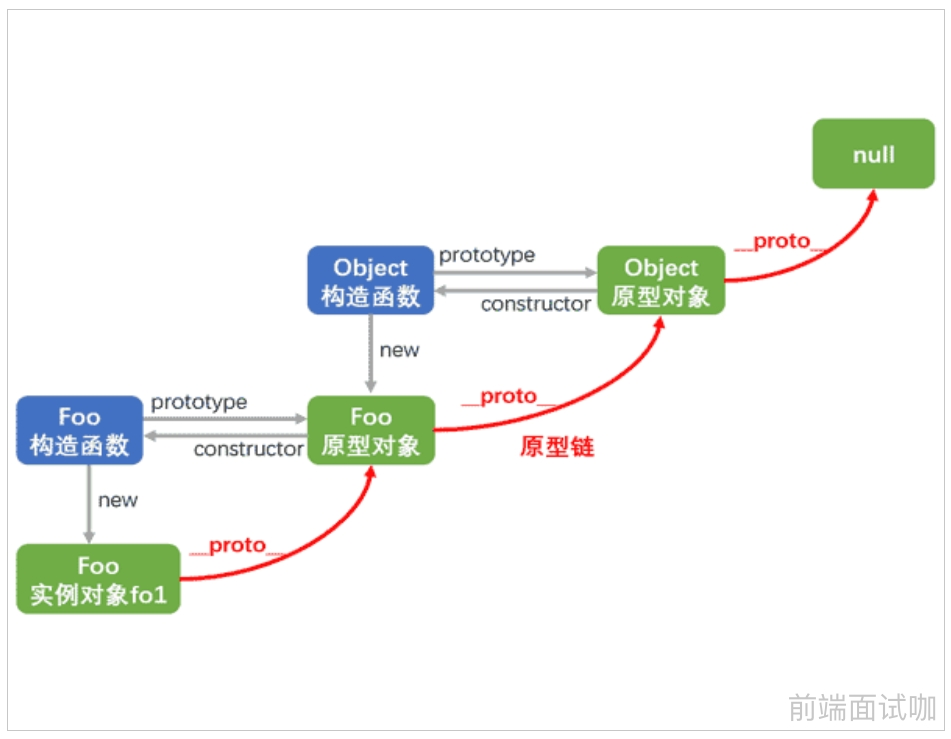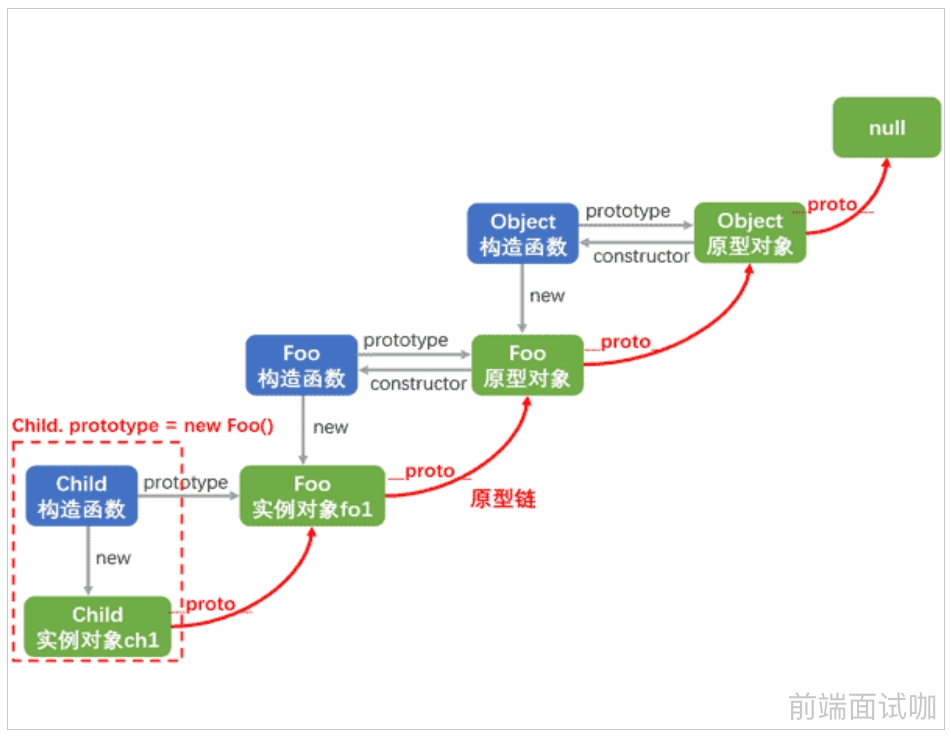js通过原型链实现函数或对象的继承

``````原型的指向可以改变

``````// 人类的构造函数
function Person(name, age) {
this.name = name
this.age = age
}
// 人类的原型的方法
Person.prototype.say = function() {
console.log('hello')
}

// 中国人的构造函数，中国人有身份证号
function Chinese(id) {
this.id = id
}
Chinese.prototype = new Person('李三', 20)
Chinese.prototype.sayChinese = function() {
console.log('我会说中文')
}

// 美国人的构造函数，美国人没有有身份证号
function American(sex) {
this.sex = sex
}
American.prototype = new Person('Jack', 18)
American.prototype.sayAmerican = function() {
console.log('我会说英文')
};
var a = new American('男')
console.log(a.name, a.age, a.sex) // Jack 18 男
a.say() // hello
a.sayAmerican() // 我会说英文``````

### 原型作用

``````function Person(name, age) {
this.name = name;
this.age = age;
this.say = function() {
console.log('hello');
}
}
p1 = new Person();
p2 = new Person();
p1.say(); // hello
p2.say(); // hello
p1.say == p2.say; // false

for(let i = 0; i < 100; i++) {
var p = new Person('Jack', 20);
p.say();
} // 会消耗大量内存``````

``````function Person(name,age) {
this.name = name;
this.age = age;
}

Person.prototype.say = function() {
console.log('hello');
}

var p1 = new Person();
var p2 = new Person();
p1.__proto__.say(); // hello
p2.__proto__.say(); // hello
console.log(p1.__proto__.say === p2.__proto__.say) // true
console.log(p1.__proto__ == Person.prototype) // true
console.log(p2.__proto__ == Person.prototype) // true``````

## JS循环机制

Javascript是一门非阻塞单线程脚本语言

1.为何单线程？2.为何非阻塞？## 同步异步

javascript实现异步的原理

​ 首先js是单线程的语言，即同一时间只能做做一件事。那js如何实现异步的，异步和单线程不是自相矛盾吗？其实，单线程和异步确实不能同时成为一个语言的特性。js选择了成为单线程的语言，所以它本身不可能是异步的，但js的宿主环境（比如浏览器，Node）是多线程的，宿主环境通过某种方式（事件驱动）使得js具备了异步的属性

​ 浏览器的内核是多线程的，它们在内核制控下相互配合以保持同步，一个浏览器至少实现三个常驻线程：javascript引擎线程，UI渲染线程，浏览器事件触发线程

### 区别

``````同步和异步最大的区别就在于：一个需要等待，一个不需要等待。
广播，就是一个异步例子，发起者不关心接收者的状态，不需要等待接收者的返回信息
电话，就是一个同步例子，发起者需要等待接收者，接通电话后，通信才开始，需要等待接收者的返回信息``````

### 处理异步的方式

``````function f2() {
console.log('hello')
}
function f1(callback){
setTimeout(function () {
callback()
}, 1000);
}
f1(f2)  // 1秒后输出 hello``````

​ 采用回调的方式，把同步操作变成了异步操作，f1不会堵塞程序运行，相当于先执行程序的主要逻辑，将耗时的操作推迟执行

​ 回调函数是异步编程最基本的方法，其优点是简单、容易理解和部署，缺点是不利于代码的阅读和维护，各个部分之间高度耦合，流程会很混乱，而且每个任务只能指定一个回调函数

``````function A(callback) {
console.log('I am A')
callback()  //调用该函数
}
function B() {
console.log('I am B')
}
A(B)
// I am A
// I am B``````

``````function f1(){
setTimeout(function(){
},5000);
}
f1() // 5秒后输出 123``````

Promise 是异步编程的一种解决方案

``new Promise( function(resolve, reject) {...})``

​ Promise 是一个构造函数，所以可以通过`new Promise()`得到一个实例对象，Promise 实例对象代表一个异步操作

​ 每当new一个Promise实例的时候，就会立即执行这个异步操作中代码，也就是说，new的时候，除了能够得到一个promise实例之外，还会立即调用我们为Promise构造函数传递的那个function，执行这个function中的异步操作代码

Promise 对象存在以下三种状态 描述
pending 初始状态
fulfilled 成功状态
rejected 失败状态

Promise 对象的初始状态是 pending，最终状态是 fulfilled 或者 rejected，其中 fulfilled 表示成功状态，rejected 表示失败状态。状态只能够由 pending 变成 fulfilled 或 rejected，当 Promise 对象的状态改变后就不可以再改变了​ 状态1：异步执行成功，需要在内部调用成功的回调函数`resolve`把结果返回给调用者

​ 状态2：异步操作失败，需要在内部调用失败的回调函数`reject` 把结果返回给调用者

``````const p = new Promise(function(resolve, reject) {
setTimeout(function() {
resolve('hello')
}, 5000)
})
p.then(function(data) {
console.log(data)
})
// 5秒后输出 hello``````

``````function getFileByPath(path) {
return new Promise(function(resolve, reject) {
fs.readFile(path, 'utf-8', (error, data) => {
if(error) return reject(error)
resolve(data)
})
})
}

// .then先执行
getFileByPath('./files/02.txt').then(
function(data) {
console.log(data)
},
funtion(error) {
console.log(error)
})``````

``````getFileByPath('./files/01.txt')
.then(function(data) {
console.log(data)
return getFileByPath('./files/02 .txt')
})
.then(function(data2) {
console.log(data2)
return getFileByPath('./files/03 .txt')
})
.then(function(data3) {
console.log(data3)
})``````

``````getFileByPath('./files/01.txt')
.then(function(data) {
console.log(data)
return getFileByPath('./files/02 .txt')
}, function(error) {
console.log(error)
return getFileByPath('./files/02 .txt')
})
.then(function(data2) {
console.log(data2)
})``````

``````getFileByPath('./files/01.txt')
.then(function(data) {
console.log(data)
return getFileByPath('./files/02 .txt')
})
.then(function(data2) {
console.log(data2)
return getFileByPath('./files/03 .txt')
})
.then(function(data3) {
console.log(data3)
})
.catch(function(error) {
console.log(error)
})
// 如果前面有任何的promise执行失败，会立即终止所有promise，并马上进入catch去处理promise中抛出的异常``````
``````const someAsyncThing = function(flag) {
return new Promise(function(resolve, reject) {
if(flag){
resolve('ok');
}else{
reject('error')
}
});
};

someAsyncThing(true).then((data)=> {
console.log('data:',data); // 输出 'ok'
}).catch((error)=>{
console.log('error:', error); // 不执行
})

someAsyncThing(false).then((data)=> {
console.log('data:',data); // 不执行
}).catch((error)=>{
console.log('error:', error); // 输出 'error'
})``````

``````let promise = new Promise(function(resolve, reject) {
console.log(1);
resolve();
console.log(2)
});

promise.then(function() {
console.log(3);
});

console.log(4);

1 2 4 3``````

## 正则表达式

### 概念

. 除了\n以外的任意的一个字符
[] 范围 [0-9] 表示：0到9之间的任意的一个数字
[a-z] 表示：所有的小写的字母中的任意的一个
[0-9a-zA-Z] 表示：所有的数字或者是字母中的一个
| 或者 [0-9]|[a-z]: 要么是一个数字，要么是一个小写字母
() 分组、提升优先级 [0-9] | ([a-z]) | [A-Z]
([0-9])([1-5])([a-z]) 三组, 从最左边开始计算
* 前面的表达式出现了0次到多次 [a-z] [0-9]* 小写字母中的任意一个 后面是要么是没有数字的,要么是多个数字的
+ 前面的表达式出现了1次到多次 [a-z] + 小写字母一个后面最少一个9,或者多个9
? 前面的表达式出现了0次到1次(阻止贪婪模式)  [a-z]? “1231234i” i可以没有
{} 限定符 : 限定前面的表达式出现的次数 {0,} 表示的是前面的表达式出现了0次到多次,和 *一样的
{1,} 表示的是前面的表达式出现了1次到多次,和 +一样的
{0,1} 表示的是前面的表达式出现了0次到1次,和 ?一样的
{5,10} 表示的是前面的表达式出现了5次到10次
{4} 前面的表达式出现了4次
{ ,10} 错误的，不能这么写
^ 以什么开始，或者是取非(取反) ^[0-9] 以数字开头
^[a-z] 以小写字母开始
[ ^ 0-9 ] 取反,非数字
[ ^ a-z ] 非小写字母
\$ 以什么结束 [0-9] [a-z]\$ 必须以小写字母结束
\d 数字中的一个 [0-9]，表示一位数字
\D 非数字 [^0-9]，除数字以外的任意字符
\s 空白符 [\t\v\n\r\f]，包含空格、水平制表、垂直制表、换行、回车、换页符
\S 非空白符 [^ \t\v\n\r\f]
\w 非特殊符号 [0-9a-zA-Z_]，表示数字、大小写字母和下划线
\W 特殊符号 [^0-9a-zA-Z_]，表示非单词字符
\b 单词的边界

(a)
(?:a)
a(?=b) 匹配a后面有b
a(?!b) 匹配a后面没有b
(?<=b)a 匹配a前面有b
(?!b)a 匹配a前面没有b

?=?!?<= **? 用于限定它前后的表达式，不能单独使用，本身没有作用

1、使用正则表达式字面量，推荐

``var reg = /ab+c/;``

2、使用RegExp对象的构造函数

``var reg = new RegExp("ab+c");``

`exec` 一个在字符串中执行查找匹配的RegExp方法，它返回一个数组（未匹配到则返回 null）。
`test` 一个在字符串中测试是否匹配的RegExp方法，它返回 true 或 false。
`match` 一个在字符串中执行查找匹配的String方法，它返回一个数组，在未匹配到时会返回 null。
`matchAll` 一个在字符串中执行查找所有匹配的String方法，它返回一个迭代器（iterator）。
`search` 一个在字符串中测试匹配的String方法，它返回匹配到的位置索引，或者在失败时返回-1。
`replace` 一个在字符串中执行查找匹配的String方法，并且使用替换字符串替换掉匹配到的子字符串。
`split` 一个使用正则表达式或者一个固定字符串分隔一个字符串，并将分隔后的子字符串存储到数组中的 `String` 方法。

`g` 全局搜索。
`i` 不区分大小写搜索。
`m` 多行搜索。
`s` 允许 `.` 匹配换行符。
`u` 使用unicode码的模式进行匹配。
`y` 执行“粘性”搜索,匹配从目标字符串的当前位置开始，可以使用y标志。

exec()

``````var reg = /d(b+)d/g;
res // ["dbbd", "bb", index: 1, input: "adbbdaaa", groups: undefined]``````

test()

``````var reg = /^\d{1,5}\$/;
var res = reg.test(12345);
res // true
var res2 = reg.test(12345789);
res2 // false``````

match()

``````var str = 'For more information, see Chapter 3.4.5.1';
var reg = /see (chapter \d+(\.\d)*)/i;
var res = str.match(reg);

console.log(res);

// logs [ 'see Chapter 3.4.5.1',
//        'Chapter 3.4.5.1',
//        '.1',
//        index: 22,

// 'see Chapter 3.4.5.1' 是整个匹配。
// 'Chapter 3.4.5.1' 被'(chapter \d+(\.\d)*)'捕获。
// '.1' 是被'(\.\d)'捕获的最后一个值。
// 'index' 属性(22) 是整个匹配从零开始的索引。
// 'input' 属性是被解析的原始字符串``````

match 使用全局（global）和忽略大小写（ignore case）标志

match 使用 global 和 ignore case 标志。A-E、a-e 的所有字母将会作为一个数组的元素返回

``````var str = 'ABCDEFGHIJKLMNOPQRSTUVWXYZabcdefghijklmnopqrstuvwxyz';
var reg = /[A-E]/gi;
var res = str.match(reg);

console.log(res);
// ['A', 'B', 'C', 'D', 'E', 'a', 'b', 'c', 'd', 'e']``````

``````var str = 'ABCDEFGHIJKLMNOPQRSTUVWXYZabcdefghijklmnopqrstuvwxyz';
var reg = /[A-E]/i;
var res = str.match(reg);
res
// ["A", index: 0, input: "ABCDEFGHIJKLMNOPQRSTUVWXYZabcdefghijklmnopqrstuvwxyz", groups: undefined]``````

``````var str1 = "NaN means not a number. Infinity contains -Infinity and +Infinity in JavaScript.",
str2 = "My grandfather is 65 years old and My grandmother is 63 years old.",
str3 = "The contract was declared null and void.";
str1.match("number");   // "number" 是字符串。返回["number"]
str1.match(NaN);        // NaN的类型是number。返回["NaN"]
str1.match(Infinity);   // Infinity的类型是number。返回["Infinity"]
str1.match(+Infinity);  // 返回["Infinity"]
str1.match(-Infinity);  // 返回["-Infinity"]
str2.match(65);         // 返回["65"]
str2.match(+65);        // 有正号的number。返回["65"]
str3.match(null);       // 返回["null"]``````

search()

``````var str = 'hello world, how are you?';
var reg = /[^\w\s]/g; // 非数字字母下划线空格
var res = str.search(reg);
res // 11 逗号的索引是11``````

replace()

``````var str = 'one dog, two dog, three dog';
var reg = /dog/g;
var res = str.replace(reg, 'cat');
res // "one cat, two cat, three cat"``````
``````var str = 'one dog, two dog, three dog';
var res = str.replace('dog', 'cat');
res // "one cat, two dog, three dog"``````

replace 第二个参数可以是个函数

``````function replacer(match, p1, p2, p3, offset, string) {
// p1 = 非数字, p2 = 数字,  p3 = 非数字、大小写字母和下划线
return [p1, p2, p3].join(' - ');
}
var newString = 'abc12345#\$*%'.replace(/([^\d]*)(\d*)([^\w]*)/, replacer);
console.log(newString);  // abc - 12345 - #\$*%``````

``````var str = 'Hello, One, Two, Three';
var res = str.replace(/two/i, 'One');
res // "Hello, One, One, Three"``````

``````var str = 'One Two Three one two three';
var res = str.replace(/one/gi, '123');
res // "123 Two Three 123 two three"``````

`\$\$` 插入一个 “\$”。
`\$&` 插入匹配的子串。
`\$` ` 插入当前匹配的子串左边的内容。
`\$'` 插入当前匹配的子串右边的内容。
`\$n` 假如第一个参数是 `RegExp`对象，并且 n 是个小于100的非负整数，那么插入第 n 个括号匹配的字符串。提示：索引是从1开始
``````var str = "John Smith";
var reg = /(\w+)\s(\w+)/;
var res = str.replace(reg, "\$2, \$1");
console.log(res); // Smith, John``````

split()

``````var str = 'one two three';
var res = str.split(' '); // ["one", "two", "three"]
res // "three"``````
``````var str = 'one two three';
var res = str.split(''); // ["o", "n", "e", " ", "t", "w", "o", " ", "t", "h", "r", "e", "e"]
 // "e"``````
``````var str = 'one two three';
var res = str.split(); // ["one two three"]``````

``````var names = "Harry Trump ;Fred Barney; Helen Rigby ; Bill Abel ;Chris Hand ";
var reg = /\s*(?:;|\$)\s*/;
var nameList = names.split(reg);

nameList // ["Harry Trump", "Fred Barney", "Helen Rigby", "Bill Abel", "Chris Hand", ""]``````

``````var str = "Hello World. How are you doing?";
var res = str.split(" ", 3);

res // ["Hello", "World.", "How"]``````

`如果 separator 包含捕获括号（capturing parentheses），则其匹配结果将会包含在返回的数组中`

``````var str = "Hello 1 word. Sentence number 2.";
var res = str.split(/(\d)/);

res // ["Hello ", "1", " word. Sentence number ", "2", "."]``````

``````lits = myString.split(['|']);

console.log(splits); //["this", "is", "a", "Test"]

const myString = 'ca,bc,a,bca,bca,bc';

const splits = myString.split(['a','b']);
// myString.split(['a','b']) is same as myString.split(String(['a','b']))

console.log(splits);  //["c", "c,", "c", "c", "c"]``````

1.两种模糊匹配

``````let r = /ab{2,5}c/g;
let s = "abc abbc abbbc abbbbbbc";
s.match(r); // ["abbc","abbbc"]``````

``````let r = /ab/g;
let s = "a0b a1b a4b";
s.match(r); // ["a1b"]``````

``````[a-z] // 匹配所有的小写字母
[A-Z] // 匹配所有的大写字母
[a-zA-Z] // 匹配所有的字母
[0-9] // 匹配所有的数字
[0-9\.\-] // 匹配所有的数字，句号和减号
[\f\r\t\n] // 匹配所有的白字符``````
``^[a-z][0-9]\$ // 匹配一个由一个小写字母和一位数字组成的字符串,比如"z2"、"t6"或"g7",但不是"ab2"、"r2d3" 或"b52"``
``^[^0-9][0-9]\$ // 这个模式与"&5"、"g7"及"-2"是匹配的，但与"12"、"66"是不匹配的``
``````[^a-z] //除了小写字母以外的所有字符
[^\\\/\^] //除了(\)(/)(^)之外的所有字符
[^\"\'] //除了双引号(")和单引号(')之外的所有字符``````

^[a-zA-Z_]\$ 所有的字母和下划线
^[[:alpha:]]{3}\$ 所有的3个字母的单词
^a\$ 字母a
^a{4}\$ aaaa
^a{2,4}\$ aa,aaa或aaaa
^a{1,3}\$ a,aa或aaa
^a{2,}\$ 包含多于两个a的字符串
^a{2,} 如：aardvark和aaab，但apple不行
\t{2} 两个制表符
.{2} 所有的两个字符
``````^[a-zA-Z0-9_]{1,}\$ //所有包含一个以上的字母、数字或下划线的字符串
^[0-9]{1,}\$ //所有的正数
^\-{0,1}[0-9]{1,}\$ //所有的整数
^\-{0,1}[0-9]{0,}\.{0,1}[0-9]{0,}\$ //所有的小数``````

``^\-?[0-9]{0,}\.?[0-9]{0,}\$``

``````^[a-zA-Z0-9_]+\$ //所有包含一个以上的字母、数字或下划线的字符串
^[0-9]+\$ //所有的正数
^\-?[0-9]+\$ //所有的整数
^\-?[0-9]*\.?[0-9]*\$ //所有的小数``````

1.通过构造函数创建对象

``````var reg=new RegExp(/\d{5}/);
var str="我的电话是10086";
var flag=reg.test(str);
console.log(flag);//true``````

2.字面量的方式创建对象

``````var reg=/\d{1,5}/;
var flag=reg.test("我的幸运数字:888");
console.log(flag);//true``````

``````console.log(/./.test("除了回车换行以为的任意字符"));//true
console.log(/.*/.test("0个到多个"));//true
console.log(/.+/.test("1个到多个"));//true
console.log(/.?/.test("哈哈"));//true
console.log(/[0-9]/.test("9527"));//true
console.log(/[a-z]/.test("what"));//true
console.log(/[A-Z]/.test("Are"));//true
console.log(/[a-zA-Z]/.test("干啥子"));//false
console.log(/[0-9a-zA-Z]/.test("9ebg"));//true
console.log(/b|(ara)/.test("abra"));//true
console.log(/[a-z]{2,3}/.test("arfsf"));//true``````

``````a = '森马男士球鞋+红色+高帮';
b = '红色+高帮';
function regStr(a, b) {
let reg = new RegExp('\\+(' + b.replace(/([+*])/g, '\\\$1') + ')\$');
return a.replace(reg, ' ' + b)
}
regStr(a, b)````````````var b = '红色+高帮';
b.replace(/[+*]/g, '\\+'); // "红色\+高帮"``````
``````a = '森马男士球鞋+红色+高帮';
b = '红色+高帮';
function regStr(a, b) {
let reg = new RegExp('\\+(' + b.replace(/([+*])/g, '\\+') + ')\$');
return a.replace(reg, ' ' + b)
}
regStr(a, b)``````

### 常见正则表达式

``````// 邮箱
var reg_email_1 = /[0-9a-zA-Z_.-]+[@][0-9a-zA-Z_.-]+([.][a-zA-Z]+){1,2}/;
var reg_email_2 = /^[a-zA-Z0-9_-]+@[a-zA-Z0-9_-]+(\.[a-zA-Z0-9_-]+)+\$/;

// 身份证：15位或者18位
var reg_idcard_1 = /([1-9][0-9]{14})|([1-9][0-9]{16}[0-9xX])/;
var reg_idcard_2 = /([1-9][0-9]{14})([0-9]{2}[0-9xX])?/;

// QQ号
var reg_qq_1 = /[1-9][0-9]{4,10}/;
var reg_qq_2 = /\d{5,11}/;

// 手机号
var reg_phone_1 = /^1\d{10}/;``````

## call()、apply()、bind()的区别

``````var name = '小王';
var age = 18;
var obj = {
name: '小张',
objAge: this.age,
myFun: function() {
console.log(this.name + '年龄：' + this.age);
}
}
obj.objAge; // 18
obj.myFun(); // 小张年龄：undefined``````
``````var a = '哈哈';
function fn() {
console.log(this.a);
}
fn(); // 哈哈``````

1. call()、apply()、bind() 都是用来重定义 this 这个对象的！

``````var name = '小王';
var age = 18;
var obj = {
name: '小张',
objAge: this.age,
myFun: function() {
console.log(this.name + '年龄：' + this.age);
}
}

var person = {
name: '哈哈',
age: 20
}

obj.myFun.call(person); // 哈哈年龄： 20
obj.myFun.apply(person); // 哈哈年龄： 20
obj.myFun.bind(person)();  // 哈哈年龄： 20``````

2. 对比call 、bind 、 apply 传参情况下

``````var name = '小王';
var age = 18;
var obj = {
name: '小张',
objAge: this.age,
myFun: function(a, b) {
console.log(this.name + '年龄：' + this.age + ' 来自' + a + '去往' + b);
}
}

var person = {
name: '哈哈',
age: 20
}

obj.myFun.call(person,'成都','上海'); // 哈哈年龄：20 来自成都去往上海
obj.myFun.apply(person,['成都','上海']); // 哈哈年龄：20 来自成都去往上海
obj.myFun.bind(person,'成都','上海')(); // 哈哈年龄：20 来自成都去往上海
obj.myFun.bind(person,['成都','上海'])(); // 哈哈年龄：20 来自成都,上海去往undefined``````

1. 三个函数的第一个参数都是 this 的指向对象
2. call 的参数是直接放进去的，第二第三第 n 个参数全都用逗号分隔（其余参数都直接传递给函数）
3. apply 的所有参数都必须放在一个数组里面传进去
4. bind 除了返回是函数以外，它的参数和 call 一样

bind

bind()方法主要就是将函数绑定到某个对象，bind()会创建一个函数，函数体内的this对象的值会被绑定到传入bind()中的第一个参数的值，例如：f.bind(obj)，实际上可以理解为obj.f()，这时f函数体内的this自然指向的是obj

``````function f1(x, y) {
console.log((x + y) + ":=====>" + this.age);
}
var ff=f1.bind(null);
ff(10,20);
//30:=====>undefined``````
``````function Person() {
this.age = 1000;
}
Person.prototype.eat = function () {
console.log("这个是吃");
};
var per = new Person();

var ff = f1.bind(per, 10, 20);
ff();
//30:=====>1000``````
``````function Person(age) {
this.age=age;
}
Person.prototype.play=function () {
console.log(this+"====>"+this.age);
};

function Student(age) {
this.age=age;
}
var per=new Person(10);
var stu=new Student(20);
//复制了一份
var ff=per.play.bind(stu);
ff();``````

## this关键字

this是在函数调用时创建的执行上下文中自动生成的一个内部属性，它会绑定（指向）一个对象。但是函数在不同的调用方式下其内部this会绑定不同对象

JavaScript 中 this 关键词指的是它所属的对象，它拥有不同的值，具体取决于它的使用位置：

``````在全局环境, this 指向顶层对象 Window

``console.log(this);   // Window``

``````// 全局函数
function fn() {
console.log(this);
}
fn(); // Window``````
``````// 局部函数
function fn() {
function f() {
console.log(this);
}
f();
}
fn(); // Window``````

``````const obj = {
a: this,  // Window
b: 10
}``````

``````const obj = {
a: this,  // Window
b: function() {
console.log(this); // obj对象本身
}
}
const fn = obj.b;
fn();  // Window``````
``````const obj = {
a: 0,
b: () => {
console.log(this);
}
}
obj.b(); // Window``````

``````function fn() {
console.log(this);
}
fn(); // Window
const obj = new fn();   //  fn{}, 即 obj``````

``````function Fn() {
this.a = 10;
let a = 100;
}
Fn.prototype.fn = function() {
console.log(this.a);
}
const obj = new Fn();
obj.fn();  // 10 说明指向了obj这个对象``````

``````const obj = {
a: 0,
b: function () {
console.log(this);      // obj
function c() {
console.log(this);    // Window
}
c();
}
}
obj.b()   ``````

call ,apply, bind

this指向传入的对象

``````const obj = {
a: 10
}
function fn() {
console.log(this)
}
fn.call(obj);      // {a: 10}
fn.apply(obj);     // {a: 10}
fn.bind(obj)();    // {a: 10}``````

``````var obj = {
a: 10,
b: function () {
console.log(this); // 指向obj
c = () => {
console.log(this); // 指向obj，指向上一层作用域中的this
}
c();
}
}
obj.b();``````

## new 操作符

new 操作符具体干了什么

``````function Foo(name) {
this.name = name;
}
var foo = new Foo('demo')``````
``````1. 开辟空间，存储创建的新的对象
2. 把this设置为当前的对象
3. 设置属性和方法的值
4. 返回当前的新的对象``````

## 闭包

1. 访问其他函数内部变量
2. 主要是用来封装变量，即把变量隐藏起来，不让外面拿到和修改，保护变量不被内存回收机制回收（缓存数据）

​ 缓存数据，优点也是缺陷，没有及时的释放

​ 由于闭包会使得函数中的变量都被保存在内存中，内存消耗很大，所以不能滥用闭包，否则会造成网页的性能问题，在IE中可能

• 返回函数
• 函数当做参数传递

``````function f1() {
var a = 10
function f2() {
console.log(a)
}
f2()
}
f1() // 10``````
``````function f1(a) {
return function () {
console.log(a)
}
}

var fn2 = f1(10)
fn2() // 10``````

``````function fn() {
var a = 10;
var obj = {
num: a
};
console.log(obj.num);
}
fn() // 10``````
``````function fn() {
var a = 20;
return {
num: a
}
}

var obj= fn()
console.log(obj.num) // 20``````

``````function fn() {
var a = 10;
a++;
console.log(a);
}
fn() // 11
fn() // 11
fn() // 11``````

``````function fn() {
var a = 10;
return function () {
a++;
return a;
}
}
var f = fn();
console.log(f()) // 11
console.log(f()) // 12
console.log(f()) // 13``````

``````function fn() {
var a = parseInt(Math.random()*10+1)
return function () {
console.log(a)
}
}
var ff=fn()
f()
f()
f()``````
``````function fn() {
var a = 100
return function () {
console.log(a)
}
}
var f = fn()
var a = 200
f() // 100``````
``````var count = 10; //全局作用域 标记为flag1
var count = 0; //函数全局作用域 标记为flag2
return function(){
count += 1; // 函数的内部作用域
console.log(count);
}
}
s(); // 1
s(); // 2``````

## 浅拷贝和深拷贝

``````var a = { count: 1, deep: { count: 2 } };
var b = Object.assign({}, a); // 该方法适用于数组或者对象
// 或者
var b = {...a};

a.count = 2;
b.count; // 1
a.deep.count = 5;
b.deep.count; // 5``````

``````function shallowCopy(source) {
let newSource = {};
for (item in source) {
if (source.hasOwnProperty(key)) {
newSource[key] = source[key];
}
}
return newSource;
}``````

``````var a = { count: 1, deep: { count: 2 } };
var b = JSON.parse(JSON.stringify(a));
b.deep.count = 20;
console.log(a); // { count: 1, deep: { count: 2 } } <-- 沒被修改
console.log(b); // { count: 1, deep: { count: 20 } }
console.log(a === b); // false
console.log(a.deep === b.deep); // false``````

``````function deepCopy(source) {
if (typeof source !== 'object') {
return '不是对象';
}
let isArray = Array.isArray(source);
let newSource = isArray ? [] : {};
for (let key in source) {
if (typeof source[key] !== 'object') {
newSource[key] = source[key];
} else {
newSource[key] = deepClone(source[key])
}
}
return newSource
}``````

``````function deepCopy(data) {
if(typeof data !== 'object') return '不是对象';
let newData = Array.isArray(data) ? [] : {};
for(let key in data) {
if(typeof data[key] !== 'object') {
newData[key] = data[key];
} else {
newData[key] = deepCopy(data[key])
}
}
return newData;
}``````

JS内存模型图• 基本数据类型的特点：直接存储在(stack)中的数据
• 引用数据类型的特点：存储的是该对象在引用地址，真实的数据存放在内存里
``````基本数据类型：number  string  boolean  null  undefined

``````// 基本数据类型，不影响其他变量
var a = 10;
var b = a;
a = 20;
console.log(b); // 10``````
``````// 引用数据类型修改，会影响其他变量
var a = { count: 10 };
var b = a
console.log(b.a) // 10
a.count = 20;
console.log(b.count) // 20``````

• 赋值操作两个变量指向同一个对象，两者互相影响
• 浅拷贝新生成一个对象，对象里面的属性是基本类型，拷贝的就是基本类型的值；如果属性是内存地址（引用类型），拷贝的就是内存地址
• 深拷贝会新生成所有对象（值对象里面层层嵌套对象），对象里面的属性是基本类型，拷贝的就是基本类型的值；如果属性是内存地址（引用类型），会新生成一个对象将内容拷贝进去

## GET和POST的区别

``````1. GET 数据传递是通过 URL 中的 ? 参数  传递到服务端的，可以在地址栏中看到传递的参数，POST 数据是通过请求体传递到服务端的，在界面上看不到

2. GET传递的数据长度有限制，因为 URL 地址长度有限（2048个字符），POST 可以提交任何类型的数据，包括文件，没有长度限制

3. GET 后退不会有影响，POST 后退会重新进行提交

4. GET 请求可以被缓存，POST 不可以被缓存

5. GET 只支持URL编码，POST 支持多种编码方式

6. GET 只支持ASCII字符，POST 没有字符类型限制

7. GET 一般用于数据查询，POST 一般用于表单提交``````

## http相关知识点

B/S结构：浏览器-服务器（Browser/Server）结构

C/S结构 ：客户端-服务器结构

``````                       权限                   路径
┌───────────────┴───────────────┐  ┌───┴────┐
└┬┘   └───────┬───────┘ └────┬────┘   └┬┘           └─────────┬─────────┘ └──┬──┘
协议        用户信息         主机名     端口                  查询参数          片段``````

HTTP 协议

General（大概）

``````Request URL: http://vmiaomalltest.vmiaomall.com/goods?id=eb6a806dcdbb4d96bd32917301125950
Request Method: GET
Status Code: 200 OK

``````Accept-Ranges: none
Connection: keep-alive
Content-Encoding: gzip
Content-Type: text/html; charset=utf-8
Date: Thu, 12 Mar 2020 03:13:58 GMT
ETag: "233be-lFVVifPpeSiCXdB4DZHhDRxugJ0"
Server: openresty/1.13.6.2
Transfer-Encoding: chunked
Vary: Accept-Encoding``````

``````Accept:text/html,application/xhtml+xml,application/xml;q=0.9,image/webp,image/apng,*/*;q=0.8,application/signed-exchange;v=b3;q=0.9

Accept-Encoding: gzip, deflate
Accept-Language: zh-CN,zh;q=0.9,en;q=0.8
Cache-Control: max-age=0
Connection: keep-alive
Host: vmiaomalltest.vmiaomall.com
User-Agent: Mozilla/5.0 (Windows NT 10.0; WOW64) AppleWebKit/537.36 (KHTML, like Gecko) Chrome/80.0.3987.100 Safari/537.36``````

Query String Parameters（请求参数）

``id: eb6a806dcdbb4d96bd32917301125950``

## HTTP状态码

200 返回正常
304 服务端资源无变化，可使用缓存资源
400 请求参数不合法
401 未认证
403 服务端禁止访问该资源
404 服务端未找到该资源
408 （请求超时） 服务器等候请求时发生超时
500 服务端异常

## 函数的节流和防抖

``    节流的意思是，规定时间内，只触发一次。比如我们设定500ms，在这个时间内，无论点击按钮多少次，它都只会触发一次。具体场景可以是抢购时候，由于有无数人 快速点击按钮，如果每次点击都发送请求，就会给服务器造成巨大的压力，但是我们进行节流后，就会大大减少请求的次数。``

``    防抖的意思是，在连续的操作中，无论进行了多长时间，只有某一次的操作后在指定的时间内没有再操作，这一次才被判定有效。具体场景可以搜索框输入关键字过程中实时 请求服务器匹配搜索结果，如果不进行处理，那么就是输入框内容一直变化，导致一直发送请求。如果进行防抖处理，结果就是当我们输入内容完成后，一定时间(比如500ms)没有再 输入内容，这时再触发请求。``

## 0.1 + 0.2 精度丢失问题

0.1和0.2在转换成二进制后会无限循环，由于标准位数的限制后面多余的位数会被截掉，此时就已经出现 了精度的损失，相加后因浮点数小数位的限制而截断的二进制数字在转换为十进制就会变成 0.30000000000000004。

``var x = 0.2 + 0.1;         // x 将是 0.30000000000000004``

``var x = (0.2 * 10 + 0.1 * 10) / 10;       // x 将是 0.3``

# 常见JS函数封装

``````// 判断对象时候为数组
function isArray(arr) {
return Object.prototype.toString.call(arr) === '[object Array]';
}

// 获取数组最大值
function max(arr) {
return Math.max.apply(null, arr);
}

// 获取数组最小值
function min(arr) {
return Math.min.apply(null, arr);
}

// 数组求和
function sum(arr) {
return arr.reduce((pre, cur) => pre + cur);
}

// 数组平均值
function average(arr) {
let sum = arr.reduce((a, b) => a + b);
return sum / arr.length;
}

// 数组去重
function uniqueArray(arr) {
let a = [];
for(let i = 0; i < arr.length; i++) {
if(a.indexOf(arr[i]) === -1) {
a.push(arr[i])
}
}
return a;
}

// 深拷贝
function deepCopy(data) {
if(typeof data !== 'object') return '不是对象';
let newData = Array.isArray(data) ? [] : {};
for(let ket in data) {
if(typeof data[key] !== 'object') {
newData[key] = data[key];
} else {
newData[key] = deepCopy(data[key]);
}
}
return newData;
}

// 数组排序，数组元素都是数字
function arrSort(arr) {
return arr.sort((a, b) => a - b);
}

// 数组排序，数组元素都是对象
function arrSort(arr, key) {
return arr.sort((i, j) => {
let a = i[key];
let b = j[key];
if(a > b) {
return 1;
} else if(a < b) {
return -1;
} else {
return 0;
}
})
}``````

# http.js

``````import axios from 'axios';

const http = axios.create({
baseUrl: 'http://vmiaomall.com'
})

// 添加请求拦截器
http.interceptors.request.use((config) => {
config.url = config.url + '.do'; // 在发送请求之前做些什么
return config;
}, function (error) {
return Promise.reject(error); // 对请求错误做些什么
});

// 添加响应拦截器
axios.interceptors.response.use((res) => {
if(res.data) {
return res.data; // 对响应数据做点什么
}
}, function (error) {
return Promise.reject(error); // 对响应错误做点什么
});

export default http``````

``````import axios from 'axios';

axios.defaults.baseURL = 'https://vmiaomall.com/api';

export default {
get(url, data = {}, options = {}) {
return new Promise((resolve, reject) => {
axios.get(url, { params: data }).then(res => {
if (res.status === 200) {
resolve(res.data)
} else {
Toast(res.data.msg)
}
}).
catch(err = >{
reject(err) let errMsg = '请求失败！请检查网络';
if (err.response) errMsg = err.response.data.msg Toast(errMsg)
})
})
},

post(url, data = {}, options = {}) {
return new Promise((resolve, reject) => {
axios.post(url, data).then(res => {
if (res.status === 200) {
resolve(res.data.msg)
} else {
Toast(res.data)
}
}).
catch(err = >{
reject(err) let errMsg = '请求失败！请检查网络';
if (err.response) errMsg = err.response.data.msg Toast(errMsg)
})
})
}
}``````

# 面试题

### var, let, const的区别

``````var
var声明变量存在变量提升，可重复声明

let

const

### null和undefined的区别

``````console.log(typeof undefined);    // "undefined"
console.log(typeof null);       // "object"
console.log(null == undefined);    // true  因为两者都默认转换成了false
console.log(null === undefined);    // false   "==="表示绝对相等，null和undefined类型是不一样的，所出“false”``````

null是一个表示”无”的对象，转为数值时为0；undefined是一个表示”无”的原始值，转为数值时为NaN

``````Number(null); // 0
5 + null; // 5

Number(undefined); // NaN
5 + undefined; // NaN``````
• `null`表示没有对象，即该处不应该有值

``````（1） 作为函数的参数，表示该函数的参数不是对象
（2） 作为对象原型链的终点
Object.getPrototypeOf(Object.prototype); // null``````
• `undefined`表示缺少值，即此处应该有值，但没有定义
``````（1）变量被声明了，但没有赋值时，就等于undefined
（2) 调用函数时，应该提供的参数没有提供，该参数等于undefined
（3）对象没有赋值的属性，该属性的值为undefined
（4）函数没有返回值时，默认返回undefined``````
``````var i;
i // undefined

function f(x){console.log(x)}
f() // undefined

var  o = new Object();
o.p // undefined

var x = f();
x // undefined``````

### 什么情况下是undefined

``````1. 变量声明了，没有赋值，结果是undefined
2. 函数没有明确返回值，如果接收了，结果也是undefined
3. 使用不存在的对象属性的返回值是undefined
4. 通过不存在的数组索引访问数组元素结果是undefined``````
``````var a;
console.log(a); // undefined``````
``````function fn(a, b) {
a + b;
}
var f = fn(1, 2);
console.log(f); // undefined``````
``````var obj = {
age: 18
}
console.log(obj.name); // undefined``````
``````var arr = [5, 3, 2, 4, 1];
arr; // undefined``````

### 几种js跨域的解决方法

• `jsonp``iframe``window.name``window.postMessage`、服务器上设置代理页面

sessionStorage 空间更大，接口丰富，独立空间，非持久化本地存储，仅仅回话级别存储，这些数据只有在同一个会话中的页面才能访问并且当会话结束后数据也随之销毁
localStorage 空间更大，接口丰富，独立空间，持久化本地存储，除非主动删除数据，否则数据永远不会过期

### setTimeout和setInterval的区别

``````setTimeout(fn, delay); // 用于延迟执行某一函数，只执行一次

setInterval(fn, delay); // 用于延迟执行某一函数，循环执行，直到取消``````

### 请用js编写一个数组去重函数

``````function uniqueArr(arr) {
let a = [];
for(let i = 0; i < arr.length; i++) {
if(a.indexOf(arr[i] == -1)) {
a.push(arr[i]);
}
}
return a;
}``````

``````function unique(arr) {
return Array.from(new Set(arr));
}``````

``````function unique(arr) {
return [...new Set(arr)];
}

var arr = [1, 2, 2, 3, 2];
var a = unique(arr);
console.log(a); // [1, 2, 3]``````

### 如何通过JS判断一个数组

instanceof

``````var arr = [];
arr instanceof Array; // true``````

constructor

`constructor`属性返回对创建此对象的数组函数的引用，就是返回对象相对应的构造函数

``````var arr = [];
arr.constructor == Array; // true``````

``````function isArray(arr) {
return Object.prototype.toString.call(arr) === '[object Array]';
}``````

ES6新增方法isArray()

``````var arr = [];
Array.isArray(arr); // true``````

### Javascript如何实现继承

``原型继承、构造继承、实例继承、拷贝继承``

### 前端性能优化

``````减少http请求

### 为什么要用 Sass/Less

``````这些 CSS 预处理语言可以让 CSS 具有一定的编程风格，在我实际的开发体验中给我带来了许多便捷的地方，并且使用他们更有利于项目的后期维护

1. 可以定义变量
2. 可以嵌套式的书写
3. 提高了样式的复用性
4. 可以定义一些函数``````

### HTML5/CSS3 新特性

HTML5

``````<header></header>
<footer></footer>
<aside></aside>
<main></main>
<nav></nav>
<section></section>
<article></article>
<canvns></canvas>
<audio></audio>``````
``````绘画 canvas

sessionStorage 的数据在浏览器关闭后自动删除

``````更多的语义化标签（header/nav/footer/section...）

Canvas
webSocket
localStorage/sessionStorage``````

CSS3

``````新的选择器（属性、伪类、伪元素）

``````新增各种css选择器

### 谈谈你对ES6的理解

``````新增模板字符串（为JavaScript提供了简单的字符串插值功能）

for-of（用来遍历数据—例如数组中的值。）
arguments 对象可被不定参数和默认参数完美代替。
ES6 将 Promise 对象纳入规范，提供了原生的 Promise 对象。

let 命令实际上就增加了块级作用域。

### 请用js去除字符串空格

``````// 去除所有空格:
str = str.replace(/\s*/g,'');

// 去除两头空格:
str = str.replace(/^\s|\s\$/g,'');

// 去除左空格：
str = str.replace( /^\s*/, '');

// 去除右空格：
str = str.replace(/(\s*\$)/g, '');``````
``````var str1 = ' a b c ';
var str2 = str1.replace(/\s*/g, '');
console.log(str2); // abc``````

trim()局限性，无法去除中间的空格

``````var str1 = ' a b c ';
var str2 = str1.trim();
console.log(str2); // a b c``````

### 什么是跨域

``````1. 网络协议不同，如http协议访问https协议
2. 端口不同，如80端口访问8080端口
3. 域名不同，如 qianduanblog.com 访问 baidu.com
4. 子域名不同，如 abc.qianduanblog.com 访问 def.qianduanblog.com
5. 域名和域名对应ip，如 www.a.com 访问 20.205.28.90``````

### 网页从输入网址到渲染完成经历了哪些过程

``````1. 域名解析
2. 发起TCP的3次握手
3. 建立TCP连接后发起http请求
4. 服务器收到请求并响应HTTP请求
5. 浏览器解析htm代码,并请求htm代码中的资源(如js、css图片等)
6. 断开TCP连接（四次挥手）
7. 浏览器对页面进行渲染呈现给用户``````

### 堆和栈的区别

``````1. 申请方式的不同，栈由系统自动分配，而堆是人为申请开辟

2. 申请大小的不同，栈获得的空间较小，而堆获得的空间较大

3. 申请效率的不同，栈由系统自动分配，速度较快，而堆一般速度比较慢

4. 存储内容的不同，栈在函数调用时，函数调用语句的下一条可执行语句的地址第一个进栈，然后函数的各个参数进栈，其中静态变量是不入栈的，而堆一般是在头部用一个字节存放堆的大小，堆中的具体内容是人为安排;

5. 底层不同，栈是连续的空间，而堆是不连续的空间``````

# 面试题库1

``````for(var i=0; i<3; i++) {
setTimeout(() => {
console.log(i);
}, 0)
}
// 打印了3个： 3``````
``````for(var i=0; i<3; i++) {
setTimeout(() => {
console.log(i);
}, 10)
}
// 打印了3个： 3 这道题涉及了异步、作用域、闭包``````
``````for(let i=0; i<3; i++) {
setTimeout(() => {
console.log(i);
}, 10)
}
// 0 1 2``````

``````var a = {};
var b = a;
b.name = "abc";
console.log(a.name); // abc``````
``````var a = {n: 1};
var b = a;
a.x = a = {n: 2};
console.log(a); // {n: 2}
console.log(b); // {n: 1, x: {n: 2}}
console.log(a.x);  // undefined
console.log(b.x);  // {n:2}

// b = a 是浅拷贝，所以在堆栈中引用的是一个对象地址
// a===>堆栈中{n:1}
// b=a
// b===>指向a中的{n:1}

a和b指向堆栈 {n: 1, x: {n: 2}}
a的输出值：{ n:2 };
b的输出值：{ n:1 , x={n:2} }``````
``````var a = 1, b = 2, c = 3;
a = b = c;
console.log(a); // 3
console.log(b); // 3
console.log(c); // 3``````

``````function fn(...a){
console.log(a);
}
fn(1, 2, 3) // [1, 2, 3]``````

``````var a = null;
console.log(typeof a);// object``````

``````var a = 10;
function fn() {
console.log(a); // undefined
var a = 20;
console.log(a); // 20

}
fn();``````

var a=’object’; js原生实现对变量a进行克隆

``````var a = '10086';
var b = a.concat();
console.log(b); // 10086``````
``````var a = 10;
var b = a;
a = 20;
console.log(b); // 10``````

``````(function(foo){
return typeof foo.bar;//"undefined"
})({foo:{bar:1}})``````

``````var arr1=[{index: 0, sort: 2}, {index: 1, sort: 3}, {index: 2, sort: 4}, {index: 3, sort: 1}]

var arr2=[{index: 0}, {index: 1, sort: 2}, {index: 2}, {index: 3, sort: 1}]``````
``````var arr1=[{index: 0, sort: 2}, {index: 1, sort: 3}, {index: 2, sort: 4}, {index: 3, sort: 1}];

var a = arr1.sort((a, b) => a.sort - b.sort );
console.log(a);
// [{index: 3, sort: 1}, {index: 0, sort: 2}, {index: 1, sort: 3}, {index: 2, sort: 4}]``````
``````var arr2=[{index: 0}, {index: 1, sort: 2}, {index: 2}, {index: 3, sort: 1}];

var a = arr2.filter(item => item.sort).sort((a, b) => a.sort - b.sort);
console.log(a);
// [{index: 3, sort: 1}, {index: 1, sort: 2}]``````

# 面试题库2

[“1”, “2”, “3”].map(parseInt)答案是多少

``['1', '2', '3'].map(parseInt); //  [1, NaN, NaN]``

`[1, NaN, NaN]`因为 `parseInt` 需要两个参数 `(val, radix)`，其中`radix` 表示解析时用的基数

`map`传了 `3``(element, index, array)`，对应的 `radix` 不合法导致解析失败

``````A. 23415
B. 54132
C. 23145
D. 15432

``````A. 12345
B. 54321
C. 43512
D. 45321

``````var num = 0;
function aa() {
var num = 1;
return function() {
return this.num;
}()
}
aa(); // 0
A. 报错
B. undefined
C. 1
D. 0``````

``````var f = function g() {
return 23;
}
typeof g(); // Uncaught ReferenceError: g is not defined
A. number
B. undefined
C. funtion
D. Error``````

``````setTimeout(function() {
var p = new Promise(function(resolve, reject) {
console.log(1);
resolve();
})
p.then(function() {
console.log(2);
})
console.log(3);
}, 1000)
// 1 3 2``````
``````var p = new Promise((resolve, reject) => {
console.log(5);
resolve();
}).then(() => {
setTimeout(function() { console.log(6) }, 0)
return 7;
}).then(v => {
console.log(v);
})
console.log(4); // 5 4 7 undefined 6``````

``````A. 通过xss(cross site script)跨站式脚本攻击
B. 通过域名DNS解析劫持
C. 通过sql注入的方式
D. 通过黑客攻击

// XSS(跨站脚本攻击)
// CSRF（跨站请求伪造）
// SQL注入
// DDOS``````

`````` A. 301 状态码是临时重定向
B. get ⽅方式只能⽀支持 ASCII 字符
C. get 在从服务器上获取资源，post 重点在向服务器发送数据
D. HTTPS 就是 HTTP 加上加密处理``````

``A. block    B. 不变     C. inline    D. inline-block``

``````A. Xml是种可扩展标记语言，格式更规范，是作为未来html的替代
B. Xml一般用于传输和存储数据，是对html的补充，两者的目的不同
C. 在JavaScript里解析和处理xml数据时，因为浏览器的不同，其做法也不同
D. 在IE浏览器里处理xml，首先需要创建ActiveXObject对象``````

# 面试题库3

JavaScript的规定，NaN表示的是非数字， 但是这个非数字也是不同的，因此，NaN 不等于 NaN，并且两个NaN永远不可能相等

``````NaN == NaN; // false
NaN === NaN; // false

let num;
console.log(num); // undefined
console.log(num + 10 === NaN); // false

let x = 0.1, y = 0.2
x + y === 0.3; // false``````
``````undefined == undefined; // true
undefined === undefined; // true

null == null; // true
null === null; // true``````

``````let num = 0;
console.log(num++); // 0
console.log(++num); // 2
console.log(num); // 2``````

``````parseInt('12'); // 12
parseInt('12a'); // 12
parseInt('b12a'); // NaN

Number('12'); // 12
Number('12a'); // NaN
Number('b12a'); // NaN``````

``````Boolean(1); // true
Boolean(0); // false
Boolean(-1); // true
Boolean('0'); // true
Boolean(undefined); // false
Boolean(null); // false
Boolean(NaN); // false
Boolean(''); // false
Boolean('hello'); // true

Boolean([]); // true
Boolean({}); // true``````

``````8 < 7 && 3 < 4; // false
-2 && 6 + 6 && null; // null
1 + 1 && 0 & 5; // 0``````

``````0 || 23; // 23
0 || false || true; // true
null || 10 < 8 || 10 + 10; // 20
null || 10 < 8 || false; // false``````

``````-1 ? 'aaa' : 'bbb'; // aaa
'0' ? 'aaa' : 'bbb'; // aaa

var n = '0';
+n ? 'aaa' : 'bbb'; // bbb

Boolean(+'0'); // false``````

``````f1();
function f1() {
console.log('aaa');
}
f1();
function f1() {
console.log('bbb');
}
f1();
// 输出结果是: bbb  bbb  bbb``````

``````function fn(a, b) {
console.log('函数1');
}
function fn() {
console.log('函数2')
}
fn(10, 20);
fn();
// 函数2
// 函数2``````

``````var a = function() {
console.log('aaa');
}
a();

var a = function() {
console.log('bbb');
}
a();
// aaa
// bbb

`上面3题解析`

``````// 示例1
var a = 1;
console.log(a); // 1
var a = 2;
console.log(a); // 2``````

``````// 示例2
a = 1;
console.log(a); // 1
var a;
console.log(a); // 1``````

``````// 示例3
console.log(a); // undefined
var a = 1;
console.log(a); // 1``````

``````// 示例1
var a;
var a; // 重复声明会被忽视
a = 1;
console.log(a); // 1
a = 2;
console.log(a); // 2

// 示例2
var a;
a = 1;
console.log(a); // 1

// 示例3
var a;
console.log(a); //undefined
a = 1;
console.log(a); // 1``````
``````// 示例4
fn(10); // 10

function fn(a) {
console.log(a);
}``````

``````{
var a = 2;
console.log(a);
let b = 3;
console.log(b);
}
console.log(a);
console.log(b);
// 2
// 3
// 2
// undefined``````

``````var a = 60;
var obj = {
a: 10,
b: this.a + 10,
fn: function() {
return this.a;
}
}
obj.a; // 10
obj.b; // 70
obj.fn(); // 10``````

``````var a = 20;
var obj = {
a: 10,
getA: function() {
return this.a;
}
}
obj.getA(); // 10
var test = obj.getA;
test(); // 20``````

``````function Person(name, age) {
this.name = name;
this.age = age;
this.say = function() {
console.log(this.name + this.age)
}
}
var a = new Person('aaa', 10);
a.say(); // aaa10

var b = new Person('bbb', 20);
b.say(); // bbb20

b = a;
b.say(); // aaa10

a = b;
a.say(); // aaa10``````

``````var age = 20;
var obj = {
age: 18,
say: () => {
console.log(this.age);
}
}
obj.say(); // 20``````

``````const person = { name: 'aaa' };
function say(age) {
return `\${this.name} is \${age}`;
}
say.call(person, 21); // "aaa is 21"
say.bind(person, 21); // f``````

``````function Person(name) {
this.name = name;
this.say = function() {
setTimeout(function() {
console.log(this.name);
this.name = 'aaa';
}, 1000)
}
}
var name = 'Cat';
var p = new Person('Tom');
console.log(this.name);
p.say();
console.log(name,'111');
// Cat
// Cat 111
// Cat 一秒后``````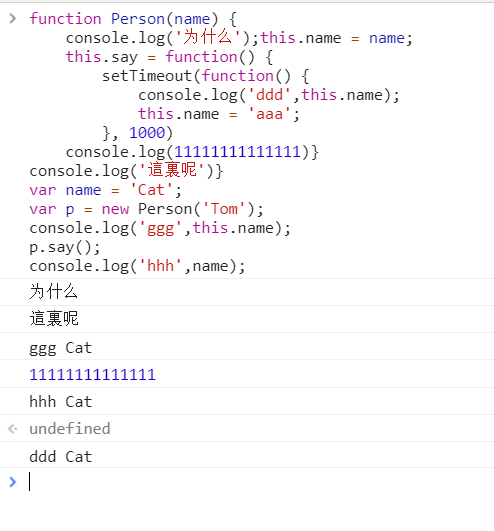``````var arr = [1, 4, 2, 4, 6, 1];
var a = [...new Set(arr)];
a; // [1, 4, 2, 6] 注意顺序``````

``````setTimeout(() => {
console.log('setTimeout');
}, 0)

new Promise((resolve, reject) => {
console.log('promise start');
resolve('promise ok');
}).then((data) => {
console.log('then result', data);
})
console.log('success');

// promise start
// success
// then result promise ok
// setTimeout 延迟一段时间``````

# 面试题库4

``````function fn() {
console.info(1);
Promise.resolve().then(function() {
console.log(2);
})
}
console.log(3);
fn();

setTimeout(function() {
console.log(4);
}, 0)

var p = new Promise(function(resolve, reject) {
console.log(5);
setTimeout(function() {
console.log(6);
}, 0)
resolve();
console.log(7);
})
p.then(function() {
console.log(8);
})
console.log(9);
// 我之前答案：3 1 2 5 7 9 8 6 4
// 正确答案： 3 1 5 7 9   2 8 4 6
// 先执行完同步任务``````

`举个例子`

``````setTimeout(function() {
console.log(1);
}, 0)
new Promise(function(resolve, reject) {
console.log(2);
resolve();
}).then(() => console.log(3))
console.log(4);
// 2 4 3 1
``````

``````var a = 10;
function fn() {
console.log(a);
let a = 20;
}
fn(); // ReferenceError: a is not defined``````

``console.log(01 + 0.2 === 0.3); // false``

``````console.log(1 + '2' + '2'); // 122
console.log(1 + + '2' + '2'); // 32
console.log(1 + - '1' + '2'); // 02
console.log(+ 1 + '1' + '2'); // 112
console.log('a' -'b' + '2'); // NaN2
console.log('a' -'b' + 2); // NaN``````
``````console.log(+ '2'); // 2
console.log(+ + '2'); // 2``````

``````Array(); // []
Array(3); // [, , ,]  定义了一个长度为3的空数组
Array(1, 2, 3); // [1, 2, 3]``````
``````Array.of(); // []
Array.of(3); // 
Array.of(1, 2, 3); // [1, 2, 3]``````

``````function Fn() {
this.name = '张三';
var age = 18;
this.sayAge = function() {
console.log('my age is ' + this.age);
}
this.sayHi = function() {
console.log('Hi' + name)
}
}
var p = new Fn();
console.log(p.name); // 张三
console.log(p.age); // undefined
p.sayAge(); // my age is undefined
p.sayHi(); // Hi

// console.log('Hi' + name) 涉及到作用域链，如果当前作用域没有这个name，那么继续往上层作用域找，直至顶层对象this``````

``````var name;
console.log(name); // 按照正常思路来说，只是声明了name变量并未赋值，所以按常理来说应该输出 undefined

// 究其原因，是因为window存在一个叫 name 的属性
// 此属性为空，实际上，开发者定义的所有变量，都会成为window的属性，如果变量没有被赋值，则该变量不会覆盖window上的同名属性

console.log(name + 'aaa'); // aaa
console.log(this.name + 'aaa'); // aaa``````

``````function fn() {
setTimeout(fn, 0)
}

function fn() {
return Promise.resolve().then(fn)
}``````

``````function fn() {
for(var i = 0; i < 10; i++) {
if(i === 3) {
return;
}
console.log(i);
}
}

fn(); // 0 1 2``````
``````function fn() {
[0,1,2,3,4,5,6,7,8,9].forEach(function(i) {
if(i === 3) {
return;
}
console.log(i);
})
}
fn(); // 0 1 2 4 5 6 7 8 9  注意没有3

// forEach中不能使用continue和break
// forEach中使用return语句的作用只能跳出当前循环，并不能跳出整个循环

// forEach 循环不能被 return 终止，其作用和 for循环中的 continue 相似 只是跳出当前循环，继续执行下一次循环，在 forEach 中也不能使用 break，continue 来跳出循环 同样会有报错``````

``````var objA = {a: 10, b: 20};
var objB = objA;
console.log(objA.a); // 10

objB.a = 30;
console.log(objA.a); // 30

setTimeout(function() {
objA.a = 10;
}, 0)

console.log(objB.a); // 30``````

``````var arr = new Set([0,1,2,3,3]);
console.log(arr); // Set(4) {0, 1, 2, 3}
console.log(arr.length); // undefined``````

# 面试题库5

HTML语义化的理解

• 什么是HTML语义化？

• 为什么要语义化?
1. 为了在没有CSS的情况下，页面也能呈现出很好地内容结构、代码结构
2. 用户体验，例如title、alt用于解释名词或解释图片信息的标签尽量填写有含义的词语、label标签的活用
3. 有利于SEO：和搜索引擎建立良好沟通，有助于爬虫抓取更多的有效信息：爬虫依赖于标签来确定上下文和各个关键字的权重
4. 方便其他设备解析（如屏幕阅读器、盲人阅读器、移动设备）以有意义的方式来渲染网页
5. 便于团队开发和维护，语义化更具可读性，遵循W3C标准的团队都遵循这个标准，可以减少差异化

<!DOCTYPE>作用？ 标准模式和兼容模式各有什么区别？

``````声明位于位于HTML文档中的第一行

DOCTYPE不存在或格式不正确会导致文档以兼容模式呈现

``````visibility: hidden;

opacity: 0;

display: none;

transform: scale(0);

height: 0; overflow: hidden;``````

get主要是用来请求数据的，post主要用来上传数据的，put主要用来修改数据的，delete主要用来删除数据的

# 面试题库6

``````for(var i = 1; i <= 5; i++) {
setTimeout(() => { console.log(i) }, i * 1000)
}
// 6``````

``````function test(x, y, z) {
console.log(test.length);
console.log(arguments.length);
console.log(arguments);
}
test(1, 2);
// 3
// 2
// undefined``````

``````(function() {
var x = y = 1;
}) ();
console.log(x, y); // Uncaught ReferenceError: x is not defined``````

``````var a = 101;
var b = a > 100 && (a = 70);
var c = (a > 50) || (a = 25);
console.log(a, b, c); // 70  70  true``````

``````1. hash 路由：
监听 url 中 hash 的变化，然后渲染不同的内容，这种路由不向服务器发送请求，不需要服务端的支持；
2. history 路由：
监听 url 中的路径变化，需要客户端和服务端共同的支持；``````

``````var obj = {
type: 'Identifier',
name: 'aaa'
}
var { type, name, value } = obj;
console.log(type, name, value); // Identifier aaa undefined``````

``````function group(arr) {
let a = [];
for(let i = 0; i < arr.length; i += 3) {
a.push(arr.slice(i, i + 3));
}
return a;
}
var arr = [1, 2, 3, 4, 5, 6, 7, 8, 9, 10];
group(arr); // [[1, 2, 3], [4, 5, 6], [7, 8, 9], ]``````

``````var arr = [{name: 'a', class: '一班'}, {name: 'c', class: '三班'}, {name: 'b', class: '二班'}, {name: 'd', class: '一班'}];
var a = arr.sort((a, b) => a.class - b.class);
a;
// 0: {name: "a", class: "一班"}
// 1: {name: "c", class: "三班"}
// 2: {name: "b", class: "二班"}
// 3: {name: "d", class: "一班"}``````

vue如何获取路由参数，如何编程式导航

``https://www.jianshu.com/p/c6492491d13f?clicktime=1577280094``

vue如何响应路由参数的变化

``https://www.cnblogs.com/restart77/p/13377257.html``

npm install –save 和 npm install –save-dev的区别

``https://blog.csdn.net/pange1991/article/details/88591837``

webpack基础

``````bundle：是由webpack打包出来的文件，

chunk：代码块，一个chunk由多个模块组合而成，用于代码的合并和分割。

module：是开发中的单个模块，在webpack的世界，一切皆模块，一个模块对应一个文件，webpack会从配置的entry中递归开始找出所有依赖的模块``````

webpack构建流程

``````1. 根据配置，识别入口文件；
2. 逐层识别模块依赖（包括 Commonjs、AMD、或 ES6 的 import 等，都会被识别和分析）；
3. Webpack 主要工作内容就是分析代码，转换代码，编译代码，最后输出代码；
4. 输出最后打包后的代码。``````

``````file-loader: 把文件输出到一个文件夹中，在代码中通过相对 URL 去引用输出的文件
style-loader: 把 CSS 代码注入到 JavaScript 中，通过 DOM 操作去加载 CSS
eslint-loader: 通过 ESLint 检查 JavaScript 代码

webpack打包是如何优化前端性能的

``````1. 压缩代码。删除多余的代码、注释、简化代码的写法等等方式。可以利用webpack的UglifyJsPlugin和ParallelUglifyPlugin来压缩JS文件， 利用cssnano（css-loader?minimize）来压缩css

3. 删除死代码（Tree Shaking）。将代码中永远不会走到的片段删除掉。可以通过在启动webpack时追加参数--optimize-minimize来实现

4. 提取公共代码。``````

# 面试题库7

``````事件委托是利用事件冒泡，只指定一个事件处理程序来管理某一类型的所有事件。

1. 考虑一个ul，在li的数量非常少的时候，为每一个li添加事件当然会使用for循环；但是数量多的时候这样做太浪费内存，长到上百上千上万的时候，为每个li添加事件就会对页面性能产生很大的影响。
2. 给一个ul里面的几个li添加了事件但是如果动态又生成了li则刚生成的li不具备事件这时就需要用到委托。

1. 性能要好
2. 针对新创建的元素，直接可以拥有事件

1. 为DOM中的很多元素绑定相同事件；
2. 为DOM中尚不存在的元素绑定事件；``````

``````var a;
console.log(typeof a); // undefined
console.log(b); // ReferenceError: b is not defined
b = 10;
console.log(typeof b);``````

``````var a = new Object();
var b  = a;
b.name = 'aaa';
console.log(a.name); // aaa``````

http和https的区别

``````1. HTTP 明文传输，数据都是未加密的，安全性较差，HTTPS（SSL+HTTP） 数据传输过程是加密的，安全性较好。

2. 使用 HTTPS 协议需要到 CA（Certificate Authority，数字证书认证机构） 申请证书，一般免费证书较少，因而需要一定费用。证书颁发机构如：Symantec、Comodo、GoDaddy 和 GlobalSign 等。

3. HTTP 页面响应速度比 HTTPS 快，主要是因为 HTTP 使用 TCP 三次握手建立连接，客户端和服务器需要交换 3 个包，而 HTTPS除了 TCP 的三个包，还要加上 ssl 握手需要的 9 个包，所以一共是 12 个包。

4. http 和 https 使用的是完全不同的连接方式，用的端口也不一样，前者是 80，后者是 443。

5. HTTPS 其实就是建构在 SSL/TLS 之上的 HTTP 协议，所以，要比较 HTTPS 比 HTTP 要更耗费服务器资源。``````

vuex刷新数据丢失怎么解决

``````1. 将vuex中的数据直接保存到浏览器缓存中（sessionStorage、localStorage、cookie）
2. 在页面刷新的时候再次请求远程数据，使之动态更新vuex数据
3. 在父页面向后台请求远程数据，并且在页面刷新前将vuex的数据先保存至sessionStorage（以防请求数据量过大页面加载时拿不到返回的数据）``````

vue首屏优化

# 面试题库8

``function Foo(``